# US20100231153A1 - Driving apparatus for three-phase AC synchronous motor - Google Patents

## Info

Publication number
US20100231153A1
US20100231153A1 US12/659,312 US65931210A US2010231153A1 US 20100231153 A1 US20100231153 A1 US 20100231153A1 US 65931210 A US65931210 A US 65931210A US 2010231153 A1 US2010231153 A1 US 2010231153A1
Authority
US
United States
Prior art keywords
phase
electric current
currents
transistors
phases
Prior art date
Legal status (The legal status is an assumption and is not a legal conclusion. Google has not performed a legal analysis and makes no representation as to the accuracy of the status listed.)
Granted
Application number
US12/659,312
Other versions
US8129932B2 (en
Inventor
Koji Sakai
Current Assignee (The listed assignees may be inaccurate. Google has not performed a legal analysis and makes no representation or warranty as to the accuracy of the list.)
Denso Corp
Original Assignee
Denso Corp
Priority date (The priority date is an assumption and is not a legal conclusion. Google has not performed a legal analysis and makes no representation as to the accuracy of the date listed.)
Filing date
Publication date
Priority to JP2009-61252 priority Critical
Priority to JP2009061252 priority
Priority to JP2009-141094 priority
Priority to JP2009141094A priority patent/JP5412974B2/en
Application filed by Denso Corp filed Critical Denso Corp
Assigned to DENSO CORPORATION reassignment DENSO CORPORATION ASSIGNMENT OF ASSIGNORS INTEREST (SEE DOCUMENT FOR DETAILS). Assignors: SAKAI, KOJI
Publication of US20100231153A1 publication Critical patent/US20100231153A1/en
Application granted granted Critical
Publication of US8129932B2 publication Critical patent/US8129932B2/en
Active legal-status Critical Current

• 230000001360 synchronised Effects 0.000 title claims abstract description 48
• 244000171263 Ribes grossularia Species 0.000 claims abstract description 1028
• 230000001264 neutralization Effects 0.000 claims abstract description 31
• 239000003990 capacitor Substances 0.000 claims description 61
• 238000010586 diagrams Methods 0.000 description 181
• 239000000969 carriers Substances 0.000 description 10
• 241000287181 Sturnus vulgaris Species 0.000 description 1
• 230000004048 modification Effects 0.000 description 1
• 238000006011 modification reactions Methods 0.000 description 1
• 239000003973 paint Substances 0.000 description 1
• 239000004065 semiconductors Substances 0.000 description 1
• 239000011734 sodium Substances 0.000 description 1

## Images

••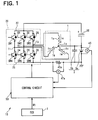•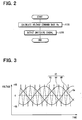•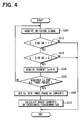••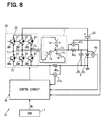•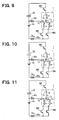•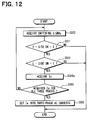•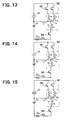•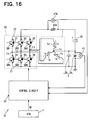•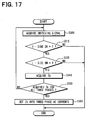•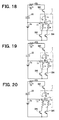•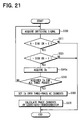•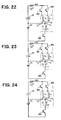•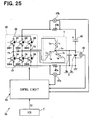•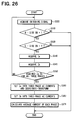•••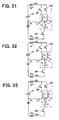•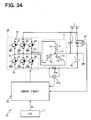•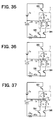•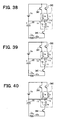•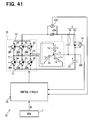••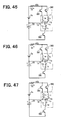•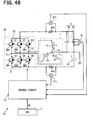•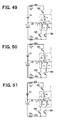•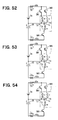•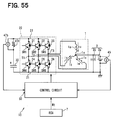••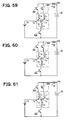•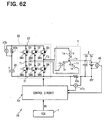•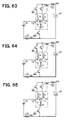•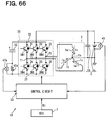•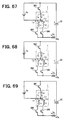•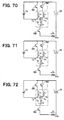••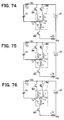•••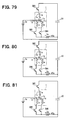•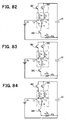••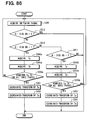••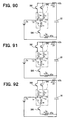•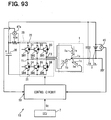•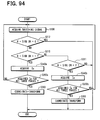••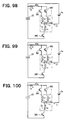•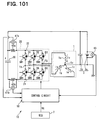•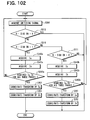•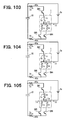•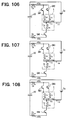## Classifications

• HELECTRICITY
• H02GENERATION; CONVERSION OR DISTRIBUTION OF ELECTRIC POWER
• H02PCONTROL OR REGULATION OF ELECTRIC MOTORS, ELECTRIC GENERATORS OR DYNAMO-ELECTRIC CONVERTERS; CONTROLLING TRANSFORMERS, REACTORS OR CHOKE COILS
• H02P27/00Arrangements or methods for the control of AC motors characterised by the kind of supply voltage
• H02P27/04Arrangements or methods for the control of AC motors characterised by the kind of supply voltage using variable-frequency supply voltage, e.g. inverter or converter supply voltage
• H02P27/06Arrangements or methods for the control of AC motors characterised by the kind of supply voltage using variable-frequency supply voltage, e.g. inverter or converter supply voltage using dc to ac converters or inverters
• H02P27/08Arrangements or methods for the control of AC motors characterised by the kind of supply voltage using variable-frequency supply voltage, e.g. inverter or converter supply voltage using dc to ac converters or inverters with pulse width modulation
• BPERFORMING OPERATIONS; TRANSPORTING
• B60VEHICLES IN GENERAL
• B60LPROPULSION OF ELECTRICALLY-PROPELLED VEHICLES; SUPPLYING ELECTRIC POWER FOR AUXILIARY EQUIPMENT OF ELECTRICALLY-PROPELLED VEHICLES; ELECTRODYNAMIC BRAKE SYSTEMS FOR VEHICLES IN GENERAL; MAGNETIC SUSPENSION OR LEVITATION FOR VEHICLES; MONITORING OPERATING VARIABLES OF ELECTRICALLY-PROPELLED VEHICLES; ELECTRIC SAFETY DEVICES FOR ELECTRICALLY-PROPELLED VEHICLES
• B60L53/00Methods of charging batteries, specially adapted for electric vehicles; Charging stations or on-board charging equipment therefor; Exchange of energy storage elements in electric vehicles
• B60L53/20Methods of charging batteries, specially adapted for electric vehicles; Charging stations or on-board charging equipment therefor; Exchange of energy storage elements in electric vehicles characterised by converters located in the vehicle
• B60L53/24Using the vehicle's propulsion converter for charging
• HELECTRICITY
• H02GENERATION; CONVERSION OR DISTRIBUTION OF ELECTRIC POWER
• H02PCONTROL OR REGULATION OF ELECTRIC MOTORS, ELECTRIC GENERATORS OR DYNAMO-ELECTRIC CONVERTERS; CONTROLLING TRANSFORMERS, REACTORS OR CHOKE COILS
• H02P2209/00Indexing scheme relating to controlling arrangements characterised by the waveform of the supplied voltage or current
• H02P2209/01Motors with neutral point connected to the power supply
• YGENERAL TAGGING OF NEW TECHNOLOGICAL DEVELOPMENTS; GENERAL TAGGING OF CROSS-SECTIONAL TECHNOLOGIES SPANNING OVER SEVERAL SECTIONS OF THE IPC; TECHNICAL SUBJECTS COVERED BY FORMER USPC CROSS-REFERENCE ART COLLECTIONS [XRACs] AND DIGESTS
• Y02TECHNOLOGIES OR APPLICATIONS FOR MITIGATION OR ADAPTATION AGAINST CLIMATE CHANGE
• Y02TCLIMATE CHANGE MITIGATION TECHNOLOGIES RELATED TO TRANSPORTATION
• Y02T10/00Road transport of goods or passengers
• Y02T10/60Other road transportation technologies with climate change mitigation effect
• Y02T10/64Electric machine technologies in electromobility
• YGENERAL TAGGING OF NEW TECHNOLOGICAL DEVELOPMENTS; GENERAL TAGGING OF CROSS-SECTIONAL TECHNOLOGIES SPANNING OVER SEVERAL SECTIONS OF THE IPC; TECHNICAL SUBJECTS COVERED BY FORMER USPC CROSS-REFERENCE ART COLLECTIONS [XRACs] AND DIGESTS
• Y02TECHNOLOGIES OR APPLICATIONS FOR MITIGATION OR ADAPTATION AGAINST CLIMATE CHANGE
• Y02TCLIMATE CHANGE MITIGATION TECHNOLOGIES RELATED TO TRANSPORTATION
• Y02T10/00Road transport of goods or passengers
• Y02T10/60Other road transportation technologies with climate change mitigation effect
• Y02T10/70Energy storage systems for electromobility, e.g. batteries
• YGENERAL TAGGING OF NEW TECHNOLOGICAL DEVELOPMENTS; GENERAL TAGGING OF CROSS-SECTIONAL TECHNOLOGIES SPANNING OVER SEVERAL SECTIONS OF THE IPC; TECHNICAL SUBJECTS COVERED BY FORMER USPC CROSS-REFERENCE ART COLLECTIONS [XRACs] AND DIGESTS
• Y02TECHNOLOGIES OR APPLICATIONS FOR MITIGATION OR ADAPTATION AGAINST CLIMATE CHANGE
• Y02TCLIMATE CHANGE MITIGATION TECHNOLOGIES RELATED TO TRANSPORTATION
• Y02T10/00Road transport of goods or passengers
• Y02T10/60Other road transportation technologies with climate change mitigation effect
• Y02T10/7072Electromobility specific charging systems or methods for batteries, ultracapacitors, supercapacitors or double-layer capacitors
• YGENERAL TAGGING OF NEW TECHNOLOGICAL DEVELOPMENTS; GENERAL TAGGING OF CROSS-SECTIONAL TECHNOLOGIES SPANNING OVER SEVERAL SECTIONS OF THE IPC; TECHNICAL SUBJECTS COVERED BY FORMER USPC CROSS-REFERENCE ART COLLECTIONS [XRACs] AND DIGESTS
• Y02TECHNOLOGIES OR APPLICATIONS FOR MITIGATION OR ADAPTATION AGAINST CLIMATE CHANGE
• Y02TCLIMATE CHANGE MITIGATION TECHNOLOGIES RELATED TO TRANSPORTATION
• Y02T90/00Enabling technologies or technologies with a potential or indirect contribution to GHG emissions mitigation
• Y02T90/10Technologies relating to charging of electric vehicles
• Y02T90/12Electric charging stations
• YGENERAL TAGGING OF NEW TECHNOLOGICAL DEVELOPMENTS; GENERAL TAGGING OF CROSS-SECTIONAL TECHNOLOGIES SPANNING OVER SEVERAL SECTIONS OF THE IPC; TECHNICAL SUBJECTS COVERED BY FORMER USPC CROSS-REFERENCE ART COLLECTIONS [XRACs] AND DIGESTS
• Y02TECHNOLOGIES OR APPLICATIONS FOR MITIGATION OR ADAPTATION AGAINST CLIMATE CHANGE
• Y02TCLIMATE CHANGE MITIGATION TECHNOLOGIES RELATED TO TRANSPORTATION
• Y02T90/00Enabling technologies or technologies with a potential or indirect contribution to GHG emissions mitigation
• Y02T90/10Technologies relating to charging of electric vehicles
• Y02T90/14Plug-in electric vehicles

## Abstract

In a driving apparatus for a three-phase synchronous motor, in which a battery is connected between a neutral point of a stator coil and a negative-pole bus, a control circuit acquires three-phase AC currents. The control circuit checks whether a neutral-point electric current is contained in an electric current detected by an electric current detecting section. Based on the check result, the control circuit checks whether electric current values for three-phases containing the neutral-point electric current are set together or electric current values for three-phases excluding the neutral-point electric current are set together to acquire the three-phase AC currents for driving the motor.

## Description

CROSS REFERENCE TO RELATED APPLICATION
• This application relates to and incorporates herein by reference Japanese Patent Applications No. 2009-61252 filed on Mar. 13, 2009 and No. 2009-141094 filed on Jun. 12, 2009.
• FIELD OF THE INVENTION
• The present invention relates to a driving apparatus for a three-phase alternating synchronous motor having a star-connected stator coil.
• BACKGROUND OF THE INVENTION
• A conventional driving apparatus for a three-phase alternating current (AC) motor includes an inverter circuit, which outputs a three-phase alternating current to a three-phase AC motor and a control circuit, which controls the inverter circuit, for example as disclosed in JP 2563226.
• Such an inverter circuit has six transistors. The six transistors will hereinafter be represented by SW1 to SW6.
• The transistors SW1 and SW4 are connectedirectly to each other between a positive-pole bus and a negative-pole bus. The transistors SW2 and SW5 are connected directly to each other between the positive-pole bus and the negative-pole bus. The transistors SW3 and SW6 are connectedirectly to each other between the positive-pole bus and the negative-pole bus. The transistors SW1, SW2 and SW3 are connected to the positive-pole bus. The transistors SW4, SW5 and SW6 are connected to the negative-pole bus.
• A common connection point of the transistors SW1 and SW4 is connected to a W-phase coil of a stator coil of the three-phase AC motor. A common connection point of the transistors SW2 and SW5 is connected to a V-phase coil of the stator coil. A common connection point of the transistors SW3 and SW6 is connected to a U-phase coil of the stator coil. The stator coil is provided by a star connection of the U-phase coil, the V-phase coil and the W-phase coil. A positive electrode of a direct current (DC) power source is connected to the positive-pole bus of the inverter circuit. A negative electrode of the DC power source is connected to the negative-pole bus.
• An electric current sensor for detecting three-phase AC currents output from the inverter circuit is connected between the positive-pole bus of the inverter circuit and the positive electrode of the DC power source. The control circuit controls the six transistors such that switching operation of the six transistors is performed on the basis of the electric current values detected by the electric current sensor. In association therewith, the inverter circuit outputs three-phase AC currents to the three-phase AC motor from the three common connection points based on a difference in voltage between the positive-pole bus and the negative-pole bus.
• When the transistors SW1, SW5 and SW6 are ON among the transistors SW1 to SW6, a W-phase electric current flowing from the inverter circuit to the W-phase coil is detected by the electric current sensor.
• When the transistors SW2, SW4 and SW6 are ON among the transistors SW1 to SW6, a V-phase electric current flowing from the inverter circuit to the V-phase coil is detected by the electric current sensor.
• When the transistors SW3, SW4 and SW5 are ON among the transistors SW1 to SW6, a U-phase electric current flowing from the inverter circuit to the U-phase coil is detected by the electric current sensor.
• The control circuit uses the W-phase electric current, the V-phase electric current and the U-phase electric current detected by the electric current sensor as described above as the three-phase AC currents and controls the three-phase AC motor through the inverter circuit based on the three-phase AC currents.
• SUMMARY OF THE INVENTION
• It is an object of the present invention to provide a driving apparatus for a three-phase AC synchronous motor having a DC power source connected between a neutral point of a stator coil and either one of a negative-pole bus or a positive-pole bus of an inverter circuit, so that the three-phase AC synchronous motor is controlled based on three-phase AC currents output from the inverter circuit to the stator coil.
• According to the present invention, an improved driving apparatus is provided for a three-phase AC synchronous motor, in which a rotor is rotated by a rotating magnetic field produced by a stator coil including three coils star-connected to a neutral point. The driving apparatus comprises an inverter circuit, a capacitor, an electric current detecting section, a current acquiring section and a control section.
• The inverter circuit includes pairs of switching elements. Each of the pairs of the switching elements is connected in series. The pairs of the switching elements are provided for respective phases and connected in parallel between a positive-pole bus and a negative-pole bus. The inverter circuit outputs three-phase AC currents to the stator coil for generating the rotating magnetic field based on two output voltages. One voltage is an output voltage from a DC power source connected between the neutral point and one of the positive-pole bus and the negative-pole bus. The other voltage is an output voltage from the capacitor. For outputting each of the three-phase AC currents, a control section turns on, at the same time, one of the switching elements connected to one of the positive-pole bus and the negative-pole bus and two of the switching elements connected to other one of the positive-pole bus and the negative-pole bus.
• The electric current detecting section detects an electric current flowing between one of the positive-pole bus and the negative-pole bus and one of the DC power source and the capacitor.
• The current acquiring section acquires, for each of the phases, an electric current containing a phase electric current output to one of the three coils as the electric current detected by the electric current detecting section. The current acquiring section acquires electric currents detected by the electric current detecting section for all three phases.
• The control section sets the electric currents for the three phases detected by the electric current detecting section and acquired by the current acquiring section together into three-phase AC currents. The control section controls, based on the three-phase AC currents, the pairs of the switching elements for the respective phases.
• BRIEF DESCRIPTION OF THE DRAWINGS
• The above and other objects, features and advantages of the present invention will become more apparent from the following detailed description made with reference to the accompanying drawings. In the drawings:
• FIG. 1 is a diagram showing the configuration of a driving apparatus in a first embodiment according to the present invention;
• FIG. 2 is a flowchart showing control processing performed by a control circuit shown in FIG. 1;
• FIG. 3 is a diagram showing a carrier wave and a voltage command wave for use in the control circuit shown in FIG. 1;
• FIG. 4 is a flowchart showing electric current calculation processing performed by the control circuit shown in FIG. 1;
• FIG. 5 is a diagram schematically showing an inverter circuit shown in FIG. 1;
• FIG. 6 is a diagram schematically showing the inverter circuit shown in FIG. 1,
• FIG. 7 is a diagram schematically showing the inverter circuit shown in FIG. 1;
• FIG. 8 is a diagram showing the configuration of a driving apparatus in a second embodiment according to the present invention;
• FIG. 9 is a diagram schematically showing an inverter circuit shown in FIG. 8;
• FIG. 10 is a diagram schematically showing the inverter circuit shown in FIG. 8;
• FIG. 11 is a diagram schematically showing the inverter circuit shown in FIG. 8;
• FIG. 12 is a diagram showing the configuration of a driving apparatus in a third embodiment according to the present invention;
• FIG. 13 is a diagram schematically showing an inverter circuit shown in FIG. 12;
• FIG. 14 is a diagram schematically showing the inverter circuit shown in FIG. 12;
• FIG. 15 is a diagram schematically showing the inverter circuit shown in FIG. 12;
• FIG. 16 is a diagram showing the configuration of a driving apparatus in a fourth embodiment according to the present invention;
• FIG. 17 is a flowchart showing electric current calculation processing performed by a control circuit shown in FIG. 16;
• FIG. 18 is a diagram schematically showing an inverter circuit shown in FIG. 16;
• FIG. 19 is a diagram schematically showing the inverter circuit shown in FIG. 16;
• FIG. 20 is a diagram schematically showing the inverter circuit shown in FIG. 16;
• FIG. 21 is a diagram showing the configuration of a driving apparatus in a fifth embodiment according to the present invention;
• FIG. 22 is a diagram schematically showing an inverter circuit shown in FIG. 21;
• FIG. 23 is a diagram schematically showing the inverter circuit shown in FIG. 21;
• FIG. 24 is a diagram schematically showing the inverter circuit shown in FIG. 21;
• FIG. 25 is a diagram showing the configuration of a driving apparatus in a sixth embodiment according to the present invention;
• FIG. 26 is a flowchart showing electric current calculation processing performed by a control circuit shown in FIG. 25;
• FIG. 27 is a diagram schematically showing an inverter circuit shown in FIG. 25;
• FIG. 28 is a diagram schematically showing the inverter circuit shown in FIG. 25;
• FIG. 29 is a diagram schematically showing the inverter circuit shown in FIG. 25;
• FIG. 30 is a diagram showing the configuration of a driving apparatus in a seventh embodiment according to the present invention;
• FIG. 31 is a diagram schematically showing an inverter circuit shown in FIG. 30.
• FIG. 32 is a diagram schematically showing the inverter circuit shown in FIG. 30;
• FIG. 33 is a diagram schematically showing the inverter circuit shown in FIG. 30;
• FIG. 34 is a diagram showing the configuration of a driving apparatus in an eighth embodiment according to the present invention;
• FIG. 35 is a diagram schematically showing an inverter circuit shown in FIG. 34;
• FIG. 36 is a diagram schematically showing the inverter circuit shown in FIG. 34;
• FIG. 37 is a diagram schematically showing the inverter circuit shown in FIG. 34;
• FIG. 38 is a diagram showing the configuration of an inverter circuit in a ninth embodiment according to the present invention;
• FIG. 39 is a diagram schematically showing the inverter circuit shown in FIG. 38;
• FIG. 40 is a diagram schematically showing the inverter circuit shown in FIG. 38;
• FIG. 41 is a diagram showing the configuration of a driving apparatus in a tenth embodiment according to the present invention;
• FIG. 42 is a diagram schematically showing an inverter circuit shown in FIG. 41;
• FIG. 43 is a diagram schematically showing the inverter circuit shown in FIG. 41;
• FIG. 44 is a diagram schematically showing the inverter circuit shown in FIG. 41;
• FIG. 45 is a schematic diagram showing an inverter circuit in an eleventh embodiment according to the present invention;
• FIG. 46 is a schematic diagram showing the inverter circuit of the eleventh embodiment;
• FIG. 47 is a schematic diagram showing the inverter circuit of the eleventh embodiment;
• FIG. 48 is a diagram showing the configuration of a driving apparatus in the twelfth embodiment according to the present invention;
• FIG. 49 is a diagram schematically showing an inverter circuit shown in FIG. 48;
• FIG. 50 is a diagram schematically showing the inverter circuit shown in FIG. 48;
• FIG. 51 is a diagram schematically showing the inverter circuit shown in FIG. 48;
• FIG. 52 is a schematic diagram showing an inverter circuit in a thirteenth embodiment according to the present invention;
• FIG. 53 is a schematic diagram showing the inverter circuit of the thirteenth embodiment;
• FIG. 54 is a schematic diagram showing the inverter circuit of the thirteenth embodiment;
• FIG. 55 is a diagram showing the configuration of a driving apparatus in a fourteenth embodiment according to the present invention;
• FIG. 56 is a diagram schematically showing an inverter circuit shown in FIG. 55;
• FIG. 57 is a diagram schematically showing the inverter circuit shown in FIG. 55;
• FIG. 58 is a diagram schematically showing the inverter circuit shown in FIG. 55;
• FIG. 59 is a schematic diagram showing an inverter circuit in a fifteenth embodiment according to the present invention;
• FIG. 60 is a schematic diagram showing the inverter circuit of the fifteenth embodiment;
• FIG. 61 is a schematic diagram showing the inverter circuit of the fifteenth embodiment;
• FIG. 62 is a diagram showing the configuration of a driving apparatus in a sixteenth embodiment according to the present invention;
• FIG. 63 is a diagram schematically showing an inverter circuit shown in FIG. 62;
• FIG. 64 is a diagram schematically showing the inverter circuit shown in FIG. 62;
• FIG. 65 is a diagram schematically showing the inverter circuit shown in FIG. 62;
• FIG. 66 is a diagram showing the configuration of a driving apparatus in a seventeenth embodiment according to the present invention;
• FIG. 67 is a diagram schematically showing an inverter circuit shown in FIG. 66;
• FIG. 68 is a diagram schematically showing the inverter circuit shown in FIG. 66;
• FIG. 69 is a diagram schematically showing the inverter circuit shown in FIG. 66;
• FIG. 70 is a schematic diagram showing an inverter circuit in an eighteenth embodiment according to the present invention;
• FIG. 71 is a schematic diagram showing the inverter circuit of the eighteenth embodiment;
• FIG. 72 is a schematic diagram showing the inverter circuit of the eighteenth embodiment;
• FIG. 73 is a diagram showing the configuration of a driving apparatus in a nineteenth embodiment according to the present invention;
• FIG. 74 is a diagram schematically showing an inverter circuit shown in FIG. 73;
• FIG. 75 is a diagram schematically showing the inverter circuit shown in FIG. 73;
• FIG. 76 is a diagram schematically showing the inverter circuit shown in FIG. 73;
• FIG. 77 is a diagram showing the configuration of a driving apparatus in a twentieth embodiment according to the present invention;
• FIG. 78 is a flowchart showing control processing performed by a control circuit shown in FIG. 77;
• FIG. 79 is a diagram schematically showing an inverter circuit shown in FIG. 77;
• FIG. 80 is a diagram schematically showing the inverter circuit shown in FIG. 77;
• FIG. 81 is a diagram schematically showing the inverter circuit shown in FIG. 77;
• FIG. 82 is a diagram schematically showing the inverter circuit shown in FIG. 77;
• FIG. 83 is a diagram schematically showing the inverter circuit shown in FIG. 77;
• FIG. 84 is a diagram schematically showing the inverter circuit shown in FIG. 77;
• FIG. 85 is a diagram showing the configuration of a driving apparatus in a twenty-first embodiment according to the present invention;
• FIG. 86 is a flowchart showing control processing performed by a control circuit shown in FIG. 85;
• FIG. 87 is a diagram schematically showing an inverter circuit shown in FIG. 85;
• FIG. 88 is a diagram schematically showing the inverter circuit shown in FIG. 85;
• FIG. 89 is a diagram schematically showing the inverter circuit shown in FIG. 85;
• FIG. 90 is a diagram schematically showing the inverter circuit shown in FIG. 85;
• FIG. 91 is a diagram schematically showing the inverter circuit shown in FIG. 85;
• FIG. 92 is a diagram schematically showing the inverter circuit shown in FIG. 85;
• FIG. 93 is a diagram showing the configuration of a driving apparatus in a twenty-second embodiment according to the present invention;
• FIG. 94 is a flowchart showing control processing performed by a control circuit shown in FIG. 93;
• FIG. 95 is a diagram schematically showing an inverter circuit shown in FIG. 93;
• FIG. 96 is a diagram schematically showing the inverter circuit shown in FIG. 93;
• FIG. 97 is a diagram schematically showing the inverter circuit shown in FIG. 93;
• FIG. 98 is a diagram schematically showing the inverter circuit shown in FIG. 93;
• FIG. 99 is a diagram schematically showing the inverter circuit shown in FIG. 93;
• FIG. 100 is a diagram schematically showing the inverter circuit shown in FIG. 93;
• FIG. 101 is a diagram showing the configuration of a driving apparatus in a twenty-third embodiment according to the present invention;
• FIG. 102 is a flowchart showing control processing performed by a control circuit shown in FIG. 101;
• FIG. 103 is a diagram schematically showing an inverter circuit shown in FIG. 101;
• FIG. 104 is a diagram schematically showing the inverter circuit shown in FIG. 101;
• FIG. 105 is a diagram schematically showing the inverter circuit shown in FIG. 101;
• FIG. 106 is a diagram schematically showing the inverter circuit shown in FIG. 101;
• FIG. 107 is a diagram schematically showing the inverter circuit shown in FIG. 101; and
• FIG. 108 is a diagram schematically showing the inverter circuit shown in FIG. 101.
• DETAILED DESCRIPTION OF THE PREFERRED EMBODIMENT First Embodiment
• Referring to FIG. 1, a driving apparatus for a three-phase AC synchronous motor according to the first embodiment of the present invention is denoted by numeral 10. The driving apparatus 10 outputs three-phase AC currents to the three-phase AC synchronous motor based on a DC voltage to drive the three-phase AC synchronous motor. A load, for example a compressor device, is connected to an output shaft of rotation of the three-phase AC synchronous motor.
• The three-phase AC synchronous motor includes a rotor (not shown), for example having a permanent magnet embedded therein and a stator coil 1 providing a rotating magnetic field to the rotor. The stator coil 1 has a U-phase coil 1 a, a V-phase coil 1 b and a, W-phase coil 1 c, which are star-connected to a neutral point 1 x. A DC power source 3 is placed between the neutral point 1 x of the stator coil 1 and the ground. The DC power source, 3 is formed of a battery 3 a and a capacitor 3 b.
• The three-phase AC synchronous motor in the present embodiment has a structure which has no sensor for detecting the position information of the rotor.
• The driving apparatus 10 includes an inverter circuit 20, a capacitor 30, a resistor 40, voltage sensors 45 and 47 a and a control circuit 50. The inverter circuit 20 outputs three-phase AC currents iu, iv and iw to the stator coil 1 based on an output voltage from the DC power source 3 and a difference in voltage between the positive electrode and the negative electrode of the capacitor 30.
• The inverter circuit 20 is formed, of transistors SW1 to SW6 and diodes D1, D2, D3, D4, D5 and D6.
• The transistors SW1 and SW4 are connected in series directly to each other between a negative-pole bus 21 and a positive-pole bus 22. The transistors SW2 and SW5 are connected in series directly to each other between the negative-pole bus 21 and the positive-pole bus 22. The transistors SW3 and SW6 are connected in series directly to each other between the negative-pole bus 21 and the positive-pole bus 22. The negative-pole bus 21 is connected to the ground.
• The transistors SW1 and SW4 are provided in a pair in association with the W-phase and a common connection point T1 of the transistors SW1 and SW4 is connected to the W-phase coil 1 c. The transistors SW2 and SW5 are provided in a pair in association with the V-phase and a common connection point T2 of the transistors SW2 and SW5 is connected to the V-phase coil 1 b. The transistors SW3 and SW6 are provided in a pair in association with the U-phase and a common connection point T3 of the transistors SW3 and SW6 is connected to the U-phase coil 1 a. The pairs of the transistors are connected in parallel between the negative-pole bus 21 and the positive-pole bus 22.
• Each of the transistors SW1 to S6 is formed by using a semiconductor transistor such as an insulated gate bipolar transistor or a field-effect transistor.
• Each of the diodes D1, D2, D3, D4, D5 and D6 is placed in inverse-parallel with the associated one of the transistors SW1 to SW6.
• The capacitor 30 provides an output voltage to the inverter circuit 20 together with the battery 3 a. The positive electrode of the capacitor 30 is connected to the positive-pole bus 22 of the inverter circuit 20. The negative electrode of the capacitor 30 is connected to the neutral point 1 x.
• The resistor 40 a is connected between the negative-pole bus 21 and a negative electrode of the battery 3 a, that is, to the ground. The resistor 40 a is used for acquiring a U-phase electric current iu, a V-phase electric current iv and a W-phase electric current iw as described below.
• The U-phase electric current iu is a phase electric current, which flows from the common connection point T3 of the transistors SW3 and SW6 to the U-phase coil 1 a. The V-phase electric current iv is a phase electric current, which flows from the common connection point T2 of the transistors SW2 and SW5 to the V-phase coil 1 b. The W-phase electric current iw is a phase electric current, which flows from the common connection point T1 of the transistors SW1 and SW4 to the W-phase coil 1 c.
• In FIG. 1, the directions of the flow of the electric currents iu, iv and iw are defined such that arrows indicate a positive direction.
• The voltage sensor 45 detects a potential difference between a positive electrode and the negative electrode of the DC power source 3.
• The voltage sensor 47 a detects a potential difference between one terminal and the other terminal of the resistor 40.
• The control circuit 50 is formed of a microcomputer and a memory and as described below, performs processing of controlling the transistors SW1 to SW6 based on the respective values detected by the sensors 45 and 47 a and the target number of revolution Nt provided by an electronic control unit (ECU) 7.
• Next, the operation in the present embodiment will be described with reference to FIGS. 2 to 7.
• The control circuit 50 performs control processing of a conventional triangular wave comparison method in accordance with a flowchart of FIG. 2. The control processing of the conventional triangular wave comparison method involves control of the inverter circuit 20 based on a comparison between a voltage command wave VS for each phase and a carrier wave Ka. FIG. 3 shows the carrier wave Ka. The carrier wave Ka is a triangular wave representing a voltage, which periodically changes from zero potential (0) toward a positive or negative side. The value detected by the voltage sensor 45 is set to a peak value VB of the carrier wave Ka.
• First, at step S100, the control circuit 50 calculates the voltage command wave for each phase for controlling an actual number of revolution (actual rotation speed) Na of the three-phase AC motor to a target number of revolution (target rotation speed) Nt of the same.
• The voltage command wave VS for each phase shows a voltage command value for each of the coils 1 a, 1 b and 1 c and is three-phase command waves formed of a U-phase command wave VU, a V-phase command wave W and a W-phase command wave VW as shown in FIG. 3. Each of the voltage command waves VU, W and VW is a sinusoidal wave representing a voltage, which periodically changes from zero potential toward a positive or negative side. As described below, the waves VU, W and VW are used in controlling the inverter circuit 20.
• A conventional voltage equation for a motor is used in the calculation of the voltage command wave for each phase. The voltage equation represents a relationship between an armature voltage for each phase produced in each of the coils 1 a, 1 b and 1 c and each of the phase electric currents iu, iv and iw. In the present embodiment, each of the phase electric currents iu, iv and iw is substituted into the voltage equation to calculate the armature voltage for each phase and the calculated armature voltage is used as the voltage command wave for each phase. The phase electric currents iu, iv and iw are acquired through electric current calculation processing described below.
• At the next step S200, a switching signal for controlling the inverter circuit 20 is acquired by using the voltage command wave VS.
• Specifically, the voltage command wave VS and the carrier wave Ka is compared for each wave to determine which of the transistors SW1 to SW6 should be turned on.
• The U-phase command wave VU is associated with the transistors SW3 and SW6. When the U-phase command wave VU is larger than the carrier wave Ka, the transistor SW3 on the side of the positive-pole bus 22, that is, the high-potential side, should be turned on and the transistor SW6 on the side of the negative-pole bus 21, that is, the low-potential side, should be turned off. When the U-phase command wave VU is smaller than the carrier wave Ka, the transistor SW3 should be turned off and the transistor SW6 should be turned on.
• The V-phase command wave W is associated with the transistors SW2 and SW5. Similarly to the case of the U-phase command wave VU, one of the transistor SW2 on the side of the positive-pole bus 22 and the transistor SW5 on the side of the negative-pole bus 21 should be turned off and the other transistor should be turned on in accordance with the result of a comparison between the V-phase command wave W and the carrier wave Ka.
• Similarly, one of the transistor SW1 on the side of the positive-pole bus 22 and the transistor SW4 on the side of the negative-pole bus 21 should be turned off and the other transistor should be turned on in accordance with the result of a comparison between the W-phase command wave VW and the carrier wave Ka.
• The control circuit 50 determines in this manner which one of the transistors SW1 to SW6 should be turned on and calculates the switching signal containing the information of the determination.
• Then, the processing returns to step S100 and repeats the processing at steps S100 and S200 to acquire a control signal for the inverter circuit 20 repeatedly.
• The switching signals acquired as described above are output to the inverter circuit 20. This causes switching operation of the transistors SW1 to SW6. As a result, three-phase AC currents are output to the stator coil 1 from the common connection points T1, T2 and T3.
• Thus, a rotating magnetic field is produced from the stator coil 1. The rotor rotates in synchronization with the rotating magnetic field.
• With the switching operation of the transistors SW4, SW5 and SW6 on the side of the negative-pole bus 21, electric charge is accumulated in the capacitor 30.
• For example, when the transistor SW4 is turned on in those transistors on the side of the negative-pole bus 21, an electric current flows from the DC power source 3 to the ground through the neutral point 1 x, the W-phase coil 1 c and the transistor SW4. At this time, energy is accumulated in the W-phase coil 1 c. Thereafter, when the transistor SW4 is turned off, an electric current based on the energy accumulated in the W-phase coil 1 c flows toward the positive-pole bus 22 through the diode D1.
• That is, the electric current flows from the W-phase coil 1 c toward the positive-pole bus 22 through the diode D1 when the transistor SW4 is turned off. The electric current flows toward the positive electrode of the capacitor 30 as a charge electric current and accumulates electric charge on the capacitor 30.
• Next, electric current calculation processing in the present embodiment will be described with reference to FIGS. 4 to 7.
• FIG. 5 shows a schematic diagram of the inverter circuit 20 when the transistors SW3, SW4 and SW5 are ON among the transistors SW1 to SW6. In FIG. 5, the transistors SW1, SW2, SW6 and the diodes D1 to D6 are not shown.
• FIG. 6 shows a schematic diagram of the inverter circuit 20 when the transistors SW2, SW4 and SW6 are ON among the transistors SW1 to SW6. In FIG. 6, the transistors SW1, SW3, SW5 and the diodes D1 to D6 are not shown.
• FIG. 7 shows a schematic diagram of the inverter circuit 20 when the transistors SW1, SW5 and SW6 are ON among the transistors SW1 to SW6. In FIG. 7, the transistors SW2, SW3, SW4 and the diodes D1 to D6 are not shown. In FIGS. 5, 6 and 7, the direction of the flow of the electric current Ia is defined such that arrows indicate the positive direction.
• FIG. 4 is a flowchart showing the electric current calculation processing. The control circuit 50 performs the electric current calculation processing in accordance with the flowchart of FIG. 4. The electric current calculation processing is repeatedly performed and the performance of the electric current calculation processing is started each time the switching signal is calculated at step S200 described above. Specifically, the electric current calculation processing is performed each time the transistor to be turned on is changed among the transistors SW1 to SW6.
• First, description is made with respect to the situation shown in FIG. 5, in which the transistors SW3, SW4 and SW5 are ON among the transistors SW1 to SW6.
• At the first step S300, the switching signal is acquired. At the next step S310, it is checked, on the basis of the switching signal whether the number of ON transistors (denoted as ON number in FIG. 4) among the transistors SW1, SW2 and SW3 (denoted as high potential side (H-side) SWs in FIG. 4) is one or not.
• It is then determined that the number of ON transistors among the transistors SW1, SW2 and SW3 is one and YES is produced at step S310. This is because only the transistor SW3 is ON (turned on) among the transistors SW1, SW2 and SW3.
• At the next step S320, it is checked, on the basis of the switching signal whether the number of ON transistors (denoted as ON number in FIG. 4) among the transistors SW4, SW5 and SW6 (denoted as low potential side (L-side) SWs in FIG. 4) is two or not.
• It is then determined that the number of ON transistors among the transistors SW4, SW5 and SW6 is two and YES is produced at step S330. This is because the transistors SW4 and SW5 are ON among the transistors SW4, SW5 and SW6.
• When the transistors SW3, SW4 and SW5 are ON among the transistors SW1 to SW6 (FIG. 5), an electric current (iv+iw) provided by adding the V-phase electric current iv to the W-phase electric current iw flows as the electric current Ia through the resistor 40 a. Assuming that an electric current flowing from the positive electrode of the battery 3 a to the neutral point 1 x of the stator coil 1 is defined as a neutral-point electric current in, the electric current (iv+iw) is equal to −(in+iu). The processing proceeds to step S340 to acquire −(in+iu) as a current value V/R provided by dividing a voltage V detected by the voltage sensor 47 a by a resistance value R of the resistor 40 a.
• At the next step S350, it is checked whether the electric current Ia flowing through the resistor 40 a has been acquired for all the three-phases or not. Since the electric current Ia has been acquired only for the U-phase at this point (FIG. 5), it is determined that the electric current Ia has not been acquired for all the three phases yet and NO is produced. Then, the processing returns to step S300.
• Next, when the transistors SW2, SW4 and SW6 are ON among the transistors SW1 to SW6 as shown in FIG. 6, the switching signal is acquired at step S300, it is determined based on the switching signal that the number of ON transistors among the transistors SW1, SW2 and SW3 is one and YES is produced at step S310.
• At the next step S320, it is determined that the number of ON transistors among the transistors SW4, SW5 and SW6 is two and YES is produced. This is because the transistors SW4 and SW6 are ON among the transistors SW4, SW5 and SW6.
• When the transistors SW2, SW4 and SW6 are ON among the transistors SW1 to SW6, an electric current (iu+iw) flows as the electric current Ia through the resistor 40 a. The electric current (iu+iw) is equal to −(in+iv). The control processing proceeds to step S340 to acquire −(in+iv) as the value V/R provided by dividing the voltage V detected by the voltage sensor 47 a by the resistance value R of the resistor 40 a.
• At the next step S350, it is checked whether the electric current Ia flowing through the resistor 40 a has been acquired for the three phases or not. Since the electric current Ia has been acquired only for the U-phase and the V-phase at this point (FIG. 6), it is determined that the electric current Ia has not been acquired for all the three phases yet and NO is produced. Then, the processing returns to step S300.
• Next, when the transistors SW1, SW5 and SW6 are ON among the transistors SW1 to SW6 as shown in FIG. 7, the switching signal is acquired at step S300 and the control processing proceeds to the next step S310. It is determined that the number of ON transistors among the transistors SW1, SW2 and SW3 is one and YES is produced at step S310. At the next step S320, it is determined that the number of ON transistors among the transistors SW4, SW5 and SW6 is two and YES is produced.
• When the transistors SW1, SW5 and SW6 are ON, an electric current (iu+iv) flows as the electric current Ia through the resistor 40 a. The electric current (iu+iv) is equal to −(in+iw). The processing proceeds to step S340 to acquire −(in+iw) as the value V/R provided by dividing the voltage V detected by the voltage sensor 47 a by the resistance value R of the resistor 40 a.
• At the next step S350, it is determined that the electric current Ia flowing-through the resistor 40 a has been acquired for all the three phases and YES is produced. The control processing proceeds to the next step S360 to set the electric current Ia for all the three phases acquired at the step S340 together into three-phase AC currents {−(in+iu), −(in+iv), −(in+iw)} of a three-phase fixed coordinate system.
• At the next step S370, the three-phase AC currents of the three-phase fixed coordinate system is coordinate-transformed to calculate the phase electric currents iu, iv, iw.
• First, assuming that a U-phase component, a V-phase component and a W-phase component of the three-phase AC currents are represented by iva, iva and iwa, respectively, the following relations (1), (2) and (3) hold:

• iua=−(in+iv)  (1)

• iva=−(in+iu)  (2)

• iwa=−(in+iw)  (3)
• Next, the three-phase AC currents iva, iva and iwa of the three-phase fixed coordinate system are substituted into the following equation 1 to calculate AC currents iα and iβ of a two-phase fixed coordinate system:
• $[ i   α i   β ] = 2 3  [ 1 - 1 / 2 - 1 / 2 0 3 / 2 - 3 / 2 ]  [ i   u i   v i   w ] [ Equation   1 ]$
• When the above relations-1, (2) and (3) are substituted into the above equation 1, the neutral-point electric currents in, which are contained in the electric currents iva, iva and iwa, cancel out each other and result in the AC currents iα and iβ, which include only the electric currents iu, iv and iw.
• The electric currents iva, iva and iwa can be transformed from the three-phase fixed coordinate system to the AC currents of the two-phase fixed coordinate system in this manner to acquire the AC currents iα and iβ of the two-phase fixed coordinate system excluding the neutral-point electric current in.
• In addition, the AC currents iα and iβ of the two-phase fixed coordinate system are transformed into electric currents of the three-phase fixed coordinate system to acquire electric currents iu, iv and iw excluding the neutral-point electric current in for each phase from the electric currents iva, iva and iwa. The electric currents iu, iv and iw thus acquired are used as the three-phase AC current for calculating the voltage command wave for each phase at step S100 described above.
• In the present embodiment described above, when the control circuit 50 has acquired the electric current Ia flowing through the resistor 40 a for the three-phases, the circuit 50 brings the electric current Ia for the three-phases together into the three-phase AC currents iva, iva and iwa of the three-phase fixed coordinate system. In addition, the control circuit 50 transforms the three-phase AC currents of the three-phase fixed coordinate system into the AC currents of the two-phase fixed coordinate system. Furthermore, the control circuit 50 transforms the AC currents of the two-phase fixed coordinate system into the three-phase AC currents of the three-phase fixed coordinate system to acquire the electric currents iu, iv and iw excluding the neutral-point electric current in for each phase from the electric currents iva, iva and iwa. Then, the control circuit 50 uses the electric currents iu, iv and iw to acquire the voltage command wave for each phase and controls the inverter circuit 20 based on the voltage command wave for each phase. In this manner, the three-phase AC synchronous motor can be controlled by using the three-phase AC currents iva, iva and iwa through the inverter circuit 20.
• In the present embodiment, the single resistor 40 a and the single voltage sensor 47 a are used in order to acquire the three-phase AC currents iva, iva and iwa.
• Some conventional driving apparatuses for three-phase AC synchronous motors employ three resistors and three voltage sensors in order to detect three-phase AC output currents from the inverter circuit 20 to the stator coil 1.
• In contrast, the single resistor 40 a and the single voltage sensor 47 a are used as described above in the present embodiment. This can reduce the number of resistors and the number of voltage sensors.
• The first embodiment described above is an example, in which the voltage command wave for each phase is calculated by using the electric currents iu, iv and iw excluding the neutral-point electric current in for each phase from the three-phase AC currents (iva, iva, iwa). Alternatively, the voltage command wave for each phase may be calculated by using three-phase AC currents (iva, iva, iwa) including the neutral-point electric current in for each phase.
• While the first embodiment described above is an example, in which the three-phase AC currents iva, iva and iwa of the three-phase fixed coordinate system are used in acquiring the Voltage command wave VS, the present invention is not limited thereto. Alternatively, AC currents of the two-phase fixed coordinate system or electric currents of a rotational coordinate system may be used to acquire the voltage command wave VS.
• Second Embodiment
• The first embodiment has shown the example, in which the three-phase AC currents iva, iva and iwa are coordinate-transformed to acquire the electric currents iu, iv and iw excluding the neutral-point electric current in for each phase from the three-phase AC currents iva, iva and iwa. Instead, in the second embodiment described below, the neutral-point electric current in is detected and the detected neutral-point electric current in is used to exclude the neutral-point electric current in for each phase from three-phase AC currents iva, iva and iwa.
• FIG. 8 shows the circuit configuration of the driving apparatus 10 in the present embodiment.
• The driving apparatus 10 in FIG. 8 is provided by adding a resistor 40 c and a voltage sensor 47 c to the driving apparatus 10 shown in FIG. 1.
• The resistor 40 c is connected between the neutral point 1 x of the stator coil 1 and the positive electrode of the battery 3 a. The resistor 40 c is used for detecting the neutral-point electric current in.
• The voltage sensor 47 c detects a potential difference between one terminal and the other terminal of the resistor 40 c.
• Next, the operation of a control circuit 50 in the present embodiment will be described with reference to FIGS. 9 to 11.
• FIG. 9 shows a schematic diagram of the inverter circuit 20 when the transistors SW3, SW4 and SW5 are ON among the transistors SW1 to SW6 and also shows the stator coil 1. FIG. 10 shows a schematic diagram of the inverter circuit 20 when the transistors SW2, SW4 and SW6 are ON and also shows the stator coil 1. FIG. 11 shows a schematic diagram of the inverter circuit 20 when the transistors SW1, SW5 and SW6 are ON and also shows the stator coil 1.
• When the transistors SW3, SW4 and SW5 are ON among the transistors SW1 to SW6 as shown in FIG. 9, the electric current −(in+iu) can be acquired as a value V/R provided by dividing the voltage V detected by the voltage sensor 47 a by the resistance value R of the resistor 40 a similarly to the first embodiment described above.
• When the transistors SW3, SW4 and SW5 are ON among the transistors SW1 to SW6 as shown in FIG. 9, the neutral-point electric current in is acquired as the value V/R provided by dividing the voltage V detected by the voltage sensor 47 c by the resistance value R of the resistor 40 c.
• Subsequently, the neutral-point electric current in is used to acquire the electric current −iu excluding the electric current −in from the electric current (in+iu) acquired as described above. In addition, the electric current −iu can be multiplied by −1 to acquire the U-phase electric current iu.
• Next, when the transistors SW2, SW4 and SW6 are ON among the transistors SW1 to SW6 as shown in FIG. 10, the electric current (in+iv) can be acquired as the value V/R provided by dividing the voltage V detected by the voltage sensor 47 a by the resistance value R of the resistor 40 a, similarly to the first embodiment described above.
• In addition, when the transistors SW2, SW4 and SW6 are ON among the transistors SW1 to SW6, the neutral-point electric current in is acquired as the value V/R provided by dividing the voltage V detected by the voltage sensor 47 c by the resistance value R of the resistor 40 c.
• Subsequently, the neutral-point electric current in is used to acquire the electric current −iv excluding the electric current −in from the electric current (in+iv) acquired as described above. In addition, the electric current −iv can be multiplied by −1 to acquire the V-phase electric current iv.
• Next, when the transistors SW1, SW5 and SW6 are ON among the transistors SW1 to SW6 as shown in FIG. 11, the electric current (in+iw) can be acquired as the value V/R provided by dividing the voltage V detected by the voltage sensor 47 a by the resistance value R of the resistor 40 a, similarly to the first embodiment described above.
• In addition, when the transistors SW1, SW5 and SW6 are ON among the transistors SW1 to SW6, the neutral-point electric current in is acquired as the value V/R provided by dividing the voltage V detected by the voltage sensor 47 c by the resistance value R of the resistor 40 c.
• Subsequently, the neutral-point electric current in is used to acquire the electric current −iw excluding the electric current −in from the electric current (in+iw) acquired as described above. In addition, the electric current −iw can be multiplied by −1 to acquire W-phase electric current iw.
• The U-phase electric current iu, the V-phase electric current iv and the W-phase electric current iw thus acquired are set together into the three-phase AC currents. The three-phase AC currents are used to calculate the voltage command wave for each phase.
• With the operation described above, the control circuit 50 can acquire the three-phase AC currents iu, iv and iw and can use the acquired three-phase AC currents iu, iv and iw to control the three-phase AC synchronous motor through the inverter circuit 20, similarly to the first embodiment described above.
• Third Embodiment
• The first and second embodiments described above have shown the examples, in which the electric current including the neutral-point electric current in added to the phase electric current is detected for each phase as the electric current Ia flowing through the resistor 40 a. Instead, in the third embodiment described below, the phase electric current is detected as the electric current Ia flowing through the resistor 40 a.
• The circuit configuration of the driving apparatus 10 in the present embodiment is identical to the circuit configuration of the driving apparatus 10 in the first embodiment described above.
• Control processing performed by the control circuit 50 in the present embodiment will be described below with reference to FIGS. 12 to 15.
• FIG. 13 shows a schematic diagram of the inverter circuit 20 when transistors SW1, SW2 and SW6 are ON among transistors SW1 to SW6. In FIG. 13, the transistors SW3, SW4, SW5 and the diodes D1 to D6 are not shown.
• FIG. 14 shows a schematic diagram of the inverter circuit 20 when the transistors SW1, SW3 and SW5 are ON among the transistors SW1 to SW6.
• In FIG. 14, the transistors SW2, SW4, SW6 and the diodes D1 to D6 are not shown.
• FIG. 15 shows a schematic diagram of the inverter circuit 20 when the transistors SW2, SW3 and SW4 are ON among the transistors SW1 to SW6. In FIG. 15, the transistors SW1, SW5, SW6 and the diodes D1 to D6 are not shown. In FIGS. 13, 14 and 15, the direction of the flow of the electric current Ia is defined such that arrows indicate a positive direction.
• FIG. 12 is a flowchart showing electric current calculation processing performed by the control circuit 50 in the present embodiment. The control circuit 50 performs the electric current calculation processing in accordance with the flowchart of FIG. 12.
• First, description is made with respect to the situation, in which the transistors SW1, SW2 and SW6 are ON among the transistors SW1 to SW6.
• At the first step S300, the switching signal is acquired. At the next step S311, it is checked, on the basis of the switching signal whether the number of ON transistors (denoted as ON number in FIG. 12) among the transistors SW1, SW2 and SW3 (denoted as high or H-side SWs in FIG. 12) is two or not.
• Since only the two transistors SW1 and SW2 are ON at this point among the transistors SW1, SW2 and SW3, it is determined that the number of ON transistors among the transistors SW1, SW2 and SW3 is two and YES is produced at step S311.
• At the next step S321, it is checked, on the basis of the switching signal whether the number of ON transistors (denoted as ON number in FIG. 12) among the transistors SW4, SW5 and SW6 (denoted as low or L-side SWs in FIG. 12) is one or not.
• Since only the transistor SW6 is ON at this point among the transistors SW4, SW5 and SW6, it is determined that the number of ON transistors among the transistors SW4, SW5 and SW6 is one and YES is produced at step S321.
• When the transistors SW1, SW2 and SW6 are ON among the transistors SW1 to SW6 (FIG. 13), the U-phase electric current iu flows as the electric current Ia through the resistor 40 a. The control processing proceeds to the next step S340 a to acquire the electric current iu as the value V/R provided by dividing the voltage V detected by the voltage sensor 47 a by the resistance value R of the resistor 40 a.
• At the next step S350, it is checked whether or not the electric current Ia flowing through the resistor 40 a has been acquired for the three-phases. Since the electric current Ia has been acquired only for the U-phase at this point, it is determined that the electric current Ia has not been acquired for the three phases yet and NO is produced.
• Then, the processing returns to step S300 to acquire the switching signal. When the transistors SW1, SW3 and SW5 are ON at this point among the transistors SW1 to SW6, it is determined that the number of ON transistors among the transistors SW1, SW2 and SW3 is two and YES is produced at the next step S311.
• Then, it is determined that only the transistor SW5 is ON among the transistors SW4, SW5 and SW6 and that the number of ON transistors among the transistors SW4, SW5 and SW6 is one and YES is produced at step S321.
• When the transistors SW1, SW3 and SW5 are ON among the transistors SW1 to SW6 (FIG. 14), the electric current iv flows as the electric current Ia through the resistor 40 a. The control processing proceeds to the next step S341 to acquire the V-phase electric current iv as the value V/R provided by dividing the voltage V detected by the voltage sensor 47 a by the resistance value R of the resistor 40 a.
• Since the electric current Ia has been acquired only for the U-phase and the V-phase, it is determined that the electric current Ia has not been acquired for the three phases yet and NO is produced at the next step S350.
• Then, the processing returns to step S300 to acquire the switching signal. When the transistors SW2, SW3 and SW4 are ON at this point among the transistors SW1 to SW6, it is determined that the number of ON transistors among the transistors SW1, SW2 and SW3 is two and YES is produced at the next step S311. It is determined that the number of ON transistors among the transistors SW4, SW5 and SW6 is one and YES is produced at the next step S321.
• When the transistors SW2, SW3 and SW4 are ON (FIG. 15), the electric current iw flows as the electric current Ia through the resistor 40 a. The control processing proceeds to the next step S340 a to acquire the W-phase electric current iw as the value V/R provided by dividing the voltage V detected by the voltage sensor 47 a by the resistance value R of the resistor 40 a.
• It is determined that the electric current Ia flowing through the resistor 40 a has been acquired for the three-phases and YES is produced at the next step S350. The control processing proceeds to the next step S360 to bring the electric current Ia for the three-phases acquired at the step S340 a together into three-phase AC currents iu, iv and iw.
• In the present embodiment described above, the control circuit 50 acquires the phase electric currents iu, iv and iw as the electric current Ia flowing through the resistor 40 a and brings the phase electric currents iu, iv and iw together into the three-phase AC currents. Therefore, similarly to the first embodiment described above, the three-phase AC synchronous motor can be controlled through the inverter circuit 20 by using the three-phase AC output from the inverter circuit 20 to the stator coil 1.
• The control circuit 50 may calculate, for each phase, average values of the phase electric currents iu, iv and iw acquired at step S360 in the third embodiment described above and the phase electric currents iu, iv and iw acquired in the first embodiment described above and may use the average values of the phase electric currents to drive the three-phase AC synchronous motor through the inverter circuit 20.
• Fourth Embodiment
• The first to third embodiments described above have shown the examples, in which the resistor 40 a is placed between the negative-pole bus 21 of the inverter circuit 20 and the negative electrode of the battery, 3 a. Instead, in the fourth embodiment described below, a resistor 40 b is placed between the positive-pole bus 22 of the inverter circuit 20 and the positive electrode of the capacitor 30 as shown in FIG. 16.
• In FIG. 16, the resistor 40 b and a voltage sensor 47 b are provided instead of the resistor 40 a and the voltage sensor 47 a in FIG. 1.
• The resistor 40 b is placed between the positive-pole bus 22 of the inverter circuit 20 and the positive electrode of the capacitor 30. The voltage sensor 47 b detects a potential difference between one terminal and the other terminal of the resistor 40 b.
• Next, control processing performed by the control circuit 50 in the present embodiment will be described with reference to FIGS. 17 to 20.
• FIG. 18 shows a schematic diagram of the inverter circuit 20 when the transistors SW3, SW4 and SW5 are ON among the transistors SW1 to SW6. In FIG. 18, the transistors SW1, SW2, SW6 and the diodes D1 to D6 are not shown.
• FIG. 19 shows a schematic diagram of the inverter circuit 20 when the transistors SW2, SW4 and SW6 are ON among the transistors SW1 to SW6. In FIG. 19, the transistors SW1, SW3, SW5 and the diodes D1 to D6 are not shown.
• FIG. 20 shows a schematic diagram of the inverter circuit 20 when the transistors SW1, SW5 and SW6 are ON among the transistors SW1 to SW6. In FIG. 20, the transistors SW2, SW3, SW4 and the diodes D1 to D6 are not shown. In FIGS. 18, 19 and 20, the direction of the flow of an electric current Ib is defined such that arrows indicate a positive direction.
• FIG. 17 is a flowchart showing electric current calculation processing performed by the control circuit 50 in the present embodiment. The control circuit 50 performs the electric current calculation processing in accordance with the flowchart of FIG. 17. In FIG. 17, reference numerals identical to those in FIG. 4 represent the same steps and the description thereof is omitted.
• At the first step S300, the switching signal is acquired. When the transistors SW3, SW4 and SW5 are ON at this point among the transistors SW1 to SW6, it is determinedthat the number of ON transistors (denoted as ON number in FIG. 17) among the transistors SW1, SW2 and SW3 (denoted as high-side SWs in FIG. 17) is one and YES is produced at the next step S310.
• It is determined that the number of ON transistors (denoted as ON number in FIG. 17) among the transistors SW4, SW5 and SW6 (denoted as lower-side SWs in FIG. 17) is two and YES is produced at the next step S320.
• When the transistors SW3, SW4 and SW5 are ON among the transistors SW1 to SW6 (FIG. 18), the control processing proceeds to the next step S345 to acquire the U-phase electric current iu as the value V/R provided by dividing the voltage V detected by the voltage sensor 47 b by the resistance value R of the resistor 40 b.
• Since the electric current Ib as the U-phase electric current iu has been acquired only for the U-phase, it is determined that the electric current Ib has not been acquired for three phases yet and NO is produced at the next step S350.
• Then, the processing returns to step S300 to acquire the switching signal. When the transistors SW2, SW4 and SW6 are ON at this point among the transistors SW1 to SW6, it is determined that the number of ON transistors of the transistors SW1, SW2 and SW3 is one and YES is produced at the next step S310. It is determined that the number of ON transistors among the transistors SW4, SW5 and SW6 is two and YES is produced at step S320.
• When the transistors SW2, SW4 and SW6 are ON (FIG. 19), the electric current iv as the electric current Ib flows through the resistor 40 b. The control processing proceeds to the next step S345 to acquire the V-phase electric current iv as the value V/R provided by dividing the voltage V detected by the voltage sensor 47 b by the resistance value R of the resistor 40 b.
• Since the electric current Ib has been acquired only for the U-phase and the V-phase, it is determined that the electric current Ib has not been acquired for the three phases yet and NO is produced at the next step S350.
• Then, the processing returns to step S300 to acquire the switching signal. When the transistors SW1, SW5 and SW6 are ON at this point among the transistors SW1 to SW6, it is determined that the number of ON transistors among the transistors SW1, SW2 and SW3 is two and YES is produced at the next step S310. It is determined that the number of ON transistors among the transistors SW4, SW5 and SW6 is one and YES is produced at the next step S320.
• When the transistors SW1, SW5 and SW6 are ON among the transistors SW1 to SW6 (FIG. 20), the electric current iw as the electric current Ib flows through the resistor 40 b. The control processing proceeds to the next step S345 to acquire the W-phase electric current iw as the value V/R provided by dividing the voltage V detected by the voltage sensor 47 b by the resistance value R of the resistor 40 b.
• It is determined that the electric current Ib flowing through the resistor 40 b has been acquired for the three-phases and YES is produced at the next step S350. The control processing proceeds to the next step S360 to bring the electric currents Ib for the three-phases acquired at the step S345 together into three-phase AC currents iu, iv and iw.
• In the present embodiment described above, the control circuit 50 detects the phase electric currents iu, iv and iw as the electric currents Ib flowing through the resistor 40 b. Thus, the three-phase AC currents iu, iv and iw are acquired similarly to the first embodiment described above. As a result, the control circuit 50 can control the three-phase AC synchronous motor based on the electric currents iu, iv and iw through the inverter circuit 20.
• Fifth Embodiment
• The fourth embodiment described above is an example, in which the phase electric current is detected for each phase as the electric current Ib flowing through the resistor 40 b. Instead, in the fifth embodiment described below, the electric current including the neutral-point electric current in added to the phase electric current is detected for each phase as the electric current Ib flowing through the resistor 40 b.
• The circuit configuration of the control circuit 50 in the present embodiment is identical to the circuit configuration in the fourth embodiment described above.
• Control processing performed by the control circuit 50 in the present embodiment will be described below with reference to FIGS. 21 to 24.
• FIG. 22 shows a schematic diagram of the inverter circuit 20 when the transistors SW1, SW2 and SW6 are ON among the transistors SW1 to SW6. In FIG. 22, the transistors SW3, SW4, SW5 and the diodes D1 to D6 are not shown.
• FIG. 23 shows a schematic diagram of the inverter circuit 20 when the transistors SW1, SW3 and SW5 are ON among the transistors SW1 to SW6. In FIG. 23, the transistors SW2, SW4, SW6 and the diodes D1 to D6 are not shown.
• FIG. 24 shows a schematic diagram of the inverter circuit 20 when the transistors SW2, SW3 and SW4 are ON among the transistors SW1 to SW6. In FIG. 24, the transistors SW1, SW5, SW6 and the diodes D1 to D6 are not shown. In FIGS. 22, 23 and 24, the direction of the flow of the electric current Ib is defined such that arrows indicate a positive direction.
• FIG. 21 is a flowchart showing electric current calculation processing performed by the control circuit 50 in the present embodiment. The control circuit 50 performs the electric current calculation processing in accordance with the flowchart of FIG. 21. In FIG. 21, reference numerals identical to those in FIG. 12 represent the same steps and the description thereof is omitted.
• First, at step S300, the switching signal is acquired. When the transistors SW1, SW2 and SW6 are ON among the transistors SW1 to SW6, it is determined that the number of ON transistors (denoted as ON number in FIG. 21) among the transistors SW1, SW2 and SW3 (denoted as high-side SWs in FIG. 21) is two and YES is produced at the next step S311.
• It is determined that the number of ON transistors (denoted as ON number in FIG. 21) among the transistors SW4, SW5 and SW6 (denoted as lower-side SWs in FIG. 21) is one and YES is produced at the next step S321.
• When the transistors SW1, SW2 and SW6 are ON among the transistors SW1 to SW6 (FIG. 22), the control processing proceeds to the next step S340 a to acquire −(in+iu) as the value V/R provided by dividing the voltage V detected by the voltage sensor 47 b by the resistance value R of the resistor 40 b.
• Since the electric current Ib has been acquired only for the U-phase, it is determined that the electric current Ib has not been acquired for three phases yet and NO is produced at the next step S350.
• Then, the processing returns to step S300 to acquire the switching signal. When the transistors SW1, SW3 and SW5 are ON at this point among the transistors SW1 to SW6, it is determined that the number of ON transistors among the transistors SW1, SW2 and SW3 is two and YES is produced at the next step S311. It is determined that only the transistor SW5 is ON among the transistors SW4, SW5 and SW6 and that the number of ON transistors among the transistors SW4, SW5 and SW6 is one and YES is produced at step 5321.
• When the transistors SW1, SW3 and SW5 are ON among the transistors SW1 to SW6 (FIG. 23), the control processing proceeds to the step S345 a to acquire −(in+iv) as the value V/R provided by dividing the voltage V detected by the voltage sensor 47 b by the resistance value R of the resistor 40 b.
• Since the electric current Ib has been acquired only for the U-phase and the V-phase, it is determined that the electric current Ib has not been acquired for the three phases yet and NO is produced at the next step S350.
• Then, the processing returns to step S300 to acquire the switching signal. When the transistors SW2, SW3 and SW4 are ON at this point among the transistors SW1 to SW6, it is determined that the number of ON transistors among the transistors SW1, SW2 and SW3 is two and YES is produced at the next step S311. It is determined that the number of ON transistors among the transistors SW4, SW5 and SW6 is one and YES is produced at the next step S321.
• When the transistors SW2, SW3 and SW4 are ON among the transistors SW1 to SW6 (FIG. 24), the control processing proceeds to the step S345 a to acquire −(in+iw) as the value V/R provided by dividing the voltage V detected by the voltage sensor 47 b by the resistance value R of the resistor 40 b.
• It is determined that the electric current. Ib flowing through the resistor 40 b has been acquired for the three-phases and YES is produced at the next step S350. The control processing proceeds to the next step S360 to set the electric currents Ib for the three-phases acquired at the step S340 a together into three-phase AC currents of the three-phase fixed coordinate system.
• At the next step S370, the three-phase AC current of the three-phase fixed coordinate system is coordinate-transformed to acquire the AC currents iα and iβ of the two-phase fixed coordinate system. In this manner, the AC currents iα and iβ of the two-phase fixed coordinate system excluding the neutral-point electric current in for each phase can be acquired, similarly to the first embodiment described above. In addition, the AC currents iα and iβ of the two-phase fixed coordinate system can be transformed into electric currents of the three-phase fixed coordinate system to acquire electric currents iu, iv and iw excluding the neutral-point electric current in for each phase from the electric currents Ib for the three-phases.
• In the present embodiment described above, the control circuit 50 acquires the electric currents Ib flowing through the resistor 40 b for the three-phases, brings the electric currents Ib for the three-phases together into the three-phase AC currents of the three-phase fixed coordinate system and coordinate-transforms the three-phase AC currents of the three-phase fixed coordinate system to acquire the three-phase AC currents iu, iv and iw. Furthermore, the control circuit 50 can control the three-phase AC synchronous motor based on the electric currents iu, iv and iw through the inverter circuit 20.
• The control circuit 50 may calculate, for each phase, average values of the phase electric currents iu, iv and iw acquired at step S370 in the fifth embodiment described above and the phase electric current iu, iv and iw acquired in the fourth embodiment described above and may use the average values of the phase electric currents to acquire the voltage command wave for each phase.
• Sixth Embodiment
• Next, description will be made with respect to the sixth embodiment, in which the first and the fourth embodiments described above are combined such that the three-phase AC synchronous motor is controlled by using average values of three-phase AC currents detected by the resistor 40 a and the voltage sensor 47 a and three-phase AC currents detected by the resistor 40 b and the voltage sensor 47 b.
• FIG. 25 shows the circuit configuration of the driving apparatus 10 according to the sixth embodiment. FIG. 25 shows the configuration, in which the resistor 40 b and the voltage sensor 47 b are added to the circuit configuration shown in FIG. 1.
• The resistor 40 b is placed between the positive-pole bus 22 of the inverter circuit 20 and the positive electrode of the capacitor 30 similarly to theourth embodiment described above. The voltage sensor 47 b detects a potential difference between one terminal and the other terminal of the resistor 40 b similarly to the fourth embodiment described above.
• Next, control processing performed by the control circuit 50 in the present embodiment will be described below with reference to FIGS. 26 to 29.
• FIG. 27 shows a schematic diagram of the inverter circuit 20 when transistors SW3, SW4 and SW5 are ON among the transistors SW1 to SW6 and also shows the stator coil 1.
• FIG. 28 shows a schematic diagram of the inverter circuit 20 when the transistors SW2, SW4 and SW6 are ON among the transistors SW1 to SW6 and also shows the stator coil 1.
• FIG. 29 shows a schematic diagram of the inverter circuit 20 when the transistors SW1, SW5 and SW6 are ON among the transistors SW1 to SW6 and also shows the stator coil 1.
• FIG. 26 is a flowchart showing electric current calculation processing performed by the control circuit 50 in the present embodiment. The control circuit 50 performs the electric current calculation processing in accordance with the flowchart of FIG. 26. In FIG. 26, reference numerals identical to those in FIG. 4 represent the same steps and the description thereof is omitted.
• First, at step S300, the switching signal is acquired. When the transistors SW3, SW4 and SW5 are ON at this point among the transistors SW1 to SW6, it is determined that the number of ON transistors (denoted as ON number in FIG. 26) among the transistors SW1, SW2 and SW3 (denoted as high-side SWs in FIG. 26) is one and YES is produced at the next step S310.
• It is determined that the number of ON transistors (denoted as ON number in FIG. 26) among the transistors SW4, SW5 and SW6 (denoted as lower-side SWs in FIG. 26) is two and YES is produced at the next step S320.
• When the transistors SW3, SW4 and SW5 are ON among the transistors SW1 to SW6 (FIG. 27), the electric current Ia is acquired as −(in+iu) at step S340. The electric current Ia is equal to the value V/R provided by dividing the voltage V detected by the voltage sensor 47 a by the resistance value R of the resistor 40 a.
• In addition, the electric current Ib is acquired as the U-phase electric current iu at step S345. The electric current Ib is equal to the value V/R provided by dividing the voltage V detected by the voltage sensor 47 b by the resistance value R of the resistor 40 b.
• Since the electric current Ia and Ib have been acquired only for the U-phase, it is determined that the electric current Ia and Ib have not been acquired for three phases yet and NO is produced at the next step S350.
• Thereafter, the processing at step S300, the YES determination at step S310, the YES determination at step S320 and the processing at steps S340 and S345 are repeated until the electric current Ia and Ib have been acquired for the three phases and thus YES is produced at step S350.
• When the transistors SW2, SW4 and SW6 are ON among the transistors SW1 to SW6 (FIG. 28), the electric current Ia is acquired as the electric current (in+iv) at step S340. In addition, the electric current Ib is acquired as the V-phase electric current iv at step S345.
• When the transistors SW1, SW5 and SW6 are ON among the transistors SW1 to SW6 as shown in FIG. 29, the electric current Ia is acquired as the electric current (in+iw) at step S340. In addition, the electric current Ib is acquired as the W-phase electric current iw at step S345.
• When the electric current Ia flowing through the resistor 40 a and the electric current Ib have been acquired for the three phases and YES is produced at step S350, the control processing proceeds to the next step S361. Similarly to the first embodiment described above, the electric current Ia for the three-phases acquired at the step S340 are set together into three-phase AC currents iva, iva and iwa of the three-phase fixed coordinate system and the three-phase AC currents iva, iva and iwa are coordinate-transformed to provide three-phase AC currents iu, iv and iw excluding neutral-point electric current in for each phase from the electric currents iva, iva and iwa.
• At the next step S362, the electric currents Ib for the three-phases acquired at the step S345 are set together into three-phase AC currents iu, iv and iw of the three-phase fixed coordinate system.
• At the next step S371, the control circuit 50 calculates, for each phase, average values of the phase electric currents iu, iv and iw acquired at step S361 and the phase electric currents iu, iv and iw acquired at step S362.
• The control circuit 50 uses the calculated average values of the phase electric currents to acquire the voltage command wave for each phase. The control circuit 50 uses the voltage command wave for each phase to acquire the switching signal similarly to the first embodiment described above. Then, the switching signals are output to the inverter circuit 20 to perform the switching operation of the transistors SW1 to SW6.
• In the present embodiment described above, the control circuit 50 calculates the average values of the three-phase AC currents acquired by using the electric current Ia flowing through the resistor 40 a and the three-phase AC currents acquired by using the electric current Ib flowing through the resistor 40 b. The circuit 50 uses the calculated average values to acquire the voltage command wave for each phase. The circuit 50 uses the voltage command wave for each phase to acquire the switching signal and outputs the acquired switching signal to the inverter circuit 20. Thus, the three-phase AC synchronous motor can be controlled through the inverter circuit 20 based on the average values.
• The sixth embodiment described above is an example, in which the three-phase AC currents iva, iva and iwa of the three-phase fixed coordinate system are coordinate-transformed to acquire the three-phase AC currents iu, iv and iw. Instead, similarly to the second embodiment described above, a resistor may be connected between the neutral point 1 x of the stator coil 1 and the positive electrode of the battery 3 a, the neutral-point electric current in flowing through the resistor may be detected and the detected neutral-point electric current in may be used to exclude the neutral-point electric current in from the three-phase AC currents iva, iva and iwa.
• Seventh Embodiment
• Next, the seventh embodiment will be described in which average values of phase electric currents are calculated on the basis of the electric current Ia flowing through the resistor 40 a and the electric current Ib flowing through the resistor 40 b at the time when two of transistors SW1, SW2 and SW3 are ON and one of transistors SW4, SW5 and SW6 is ON.
• The circuit configuration of a control circuit 50 in the present embodiment is identical to the circuit configuration of the control circuit 50 in the sixth embodiment described above.
• Next, control processing performed by the control circuit 50 in the present embodiment will be described with reference to FIGS. 30 to 33.
• FIG. 31 shows a schematic diagram of the inverter circuit 20 when transistors SW1, SW2 and SW6 are ON among the transistors SW1 to SW6. FIG. 32 shows a schematic diagram of the inverter circuit 20 when the transistors SW1, SW3 and SW5 are ON. FIG. 33 shows a schematic diagram of the inverter circuit 20 when the transistors SW2, SW3 and SW4 are ON.
• FIG. 30 is a flowchart showing electric current calculation processing performed by the control circuit 50 in the present embodiment. The control circuit 50 performs the electric current calculation processing in accordance with the flowchart of FIG. 30. In FIG. 30, reference numerals identical to those in FIG. 12 represent the same steps and the description thereof is omitted.
• First, at step S300, the switching signal is acquired. When the transistors SW1, SW2 and SW6 are ON at this point among the transistors SW1 to SW6, it is determined that the number of ON transistors (denoted as ON number in FIG. 30) among the transistors SW1, SW2 and SW3 (denoted as high-side SWs in FIG. 30) is two and YES is produced at the next step S311.
• It is determined that the number of ON transistors (denoted as ON number in FIG. 30) among the transistors SW4, SW5 and SW6 (denoted as lower-side SWs in FIG. 30) is one and YES is produced at the next step S321.
• When the transistors SW1, SW2 and SW6 are ON among the transistors SW1 to SW6 (FIG. 31), an electric current Ia is acquired as a U-phase electric current iu at step 340 a. The electric current Ia is equal to a value V/R provided by dividing the voltage V detected by the voltage sensor 47 a by the resistance value R of the resistor 40 a.
• At the next step S345 a, the electric current Ib is acquired as the electric current (in+iu). The electric current Ib is equal to the value V/R provided by dividing the voltage V detected by the voltage sensor 47 b by the resistance value R of the resistor 40 b. Since the electric current Ia and Ib have been acquired only for the U-phase, it is determined that the electric current Ia and Ib have not been acquired for three phases yet and NO is produced at the next step S350.
• Thereafter, the processing at step S300, the YES determination at step S311 and step S321 and the processing at steps S340 a and S345 a are repeated until the electric current Ia and Ib have been acquired for the three-phases and thus YES is produced at step S350. When the transistors SW1, SW3 and SW5 are ON among the transistors SW1 to SW6 (FIG. 32), the electric current Ia is acquired as the V-phase electric current iv at step S340 a.
• At the next step S345 a, the electric current Ib is acquired as the electric current (iu+iw).
• When the transistors SW2, SW3 and SW4 are ON among the transistors SW1 to SW6 (FIG. 33), the control processing proceeds to step S340 a to acquire the electric current Ia as the W-phase electric current iw. At the next step S234 a, the electric current Ib is acquired as the electric current (in+iw).
• It is determined that the electric current Ia and Ib flowing through the resistor 40 a have been acquired for the three-phases and YES is produced at the next step S350. The control circuit 50 then proceeds to the next step S363 to bring the electric current Ia for the three-phases acquired at the step S340 together into three-phase AC currents iu, iv and iw of the three-phase fixed coordinate system.
• At the next step S364, similarly to the fifth embodiment described above, the control circuit 50 brings the electric currents Ib for the three-phases acquired at the step S345 a together into three-phase AC currents iva, iva and iwa of the three-phase fixed coordinate system and coordinate-transforms the three-phase AC currents iva, iva and iwa to acquire three-phase AC currents iu, iv and iw excluding the neutral-paint electric current in for each phase from the electric currents iva, iva and iwa.
• At the next step S371, the control circuit 50 calculates, for each phase, average values of the three-phase AC currents iu, iv and iw acquired at the step S363 and the three-phase AC currents iu, iv and iw acquired at the step S364.
• Specifically, the control circuit 50 calculates, for each phase, the average values of the three-phase AC currents iu, iv and iw acquired on the basis of the electric current Ia flowing through the resistor 40 a and the three-phase AC currents iu, iv and iw acquired on the basis of the electric currents Ib flowing through the resistor 40 b. Then, the control circuit 50 uses the calculated average to acquire the voltage command wave for each phase. The control circuit 50 uses the voltage command wave to acquire the switching signal and outputs the switching signal to the inverter circuit 20 to perform switching operation of the transistors SW1 to SW6.
• In the present embodiment described above, the control circuit 50 can control the three-phase AC synchronous motor through the inverter circuit 20 by using the average values of the three-phase AC currents iu, iv and iw acquired on the basis of the electric current Ia flowing through the resistor 40 a and the three-phase AC currents iu, iv and iw acquired on the basis of the electric currents Ib flowing through the resistor 40 b, similarly to the sixth embodiment described above.
• Alternatively, the control circuit 50 may prepare the two three-phase AC currents acquired by using the resistors 40 a and 40 b in the sixth embodiment described above and the two three-phase AC currents acquired by using the resistors 40 a and 40 b in the seventh embodiment described above, may calculate the average values for each phase of the prepared four three-phase AC currents and may use the calculated average value for each phase to control the three-phase AC synchronous motor through the inverter circuit 20.
• Eighth Embodiment
• The first embodiment described above is an example, in which the battery 3 a is connected between the negative-pole bus 21 and the neutral point 1 x of the stator coil 1. Instead, in the eighth embodiment described below, the DC power source 3 is connected between the positive-pole bus 22 and the neutral point 1 x of the stator coil 1.
• FIG. 34 shows the circuit configuration of the driving apparatus 10 according to the present embodiment. The driving apparatus 10 of the present embodiment has the same circuit configuration as that in FIG. 1 except for the placement of the DC power source 3 and the capacitor 30.
• The DC power source 3 of the present embodiment is connected between the positive-pole bus 22 and the neutral point 1 x of the stator coil 1. The capacitor 30 is connected between the negative-pole bus 21 and the neutral point 1 x of the stator coil 1.
• Next, the operation in the present embodiment will be described.
• The control circuit 50 of the present embodiment performs the electric current calculation processing in accordance with the flowchart of FIG. 4, similarly to the first embodiment described above. Thus, the outline of the electric current calculation processing performed by the control circuit 50 will be described below with reference to FIGS. 35 to 37.
• FIG. 35 shows a schematic diagram of the inverter circuit 20 when the transistors SW3, SW4 and SW5 are ON. FIG. 36 shows a schematic diagram of the inverter circuit 20 when the transistors SW2, SW4 and SW6 are ON. FIG. 37 shows a schematic diagram of the inverter circuit 20 when the transistors SW1, SW5 and SW6 are ON.
• First, when the transistors SW3, SW4 and SW5 are ON among the transistors SW1 to SW6 (FIG. 35), the electric current Ia is acquired as (in+iu).
• Next, when the transistors SW2, SW4 and SW6 are ON among the transistors SW1 to SW6 (FIG. 36), the electric current Ia is acquired as (in+iv).
• Next, when the transistors SW1, SW5 and SW6 are ON among the transistors SW1 to SW6 (FIG. 37), the electric current Ia is acquired as (in+iw).
• The currents (in+iu), (in+iv) and (in+iw) thus acquired are set together into the three-phase AC currents of the three-phase fixed coordinate system. In addition, similarly to the first embodiment described above, the three-phase AC currents of the three-phase fixed coordinate system are coordinate-transformed to acquire three-phase AC currents iu, iv and iw excluding the neutral-point electric current in for each phase and the three-phase AC synchronous motor is controlled through the inverter circuit 20 based on the three-phase AC currents, currents iu, iv and iw.
• The eighth embodiment is an example, in which the three-phase AC currents iva, iva and iwa of the three-phase fixed coordinate system containing the electric currents flowing through the neutral point 1 x are coordinate-transformed to acquire the three-phase AC currents iu, iv and iw. Instead, a resistor may be connected between the neutral point 1 x of the stator coil 1 and the positive electrode of the battery 3 a, the neutral-point electric current in flowing through the resistor may be detected and the detected neutral-point electric current in may be used to exclude the neutral-point electric current in from the three-phase AC currents iva, iva and iwa, similarly to the second embodiment.
• Ninth Embodiment
• The eighth embodiment described above is an example, in which the electric current including the neutral-point electric current in added to the phase electric current is detected for each phase as the electric current Ia flowing through the resistor 40 a. Instead, in the ninth embodiment described below, the phase electric current is detected as the electric current Ia flowing through the resistor 40 a.
• The circuit configuration of the driving apparatus 10 of the present embodiment is identical to the circuit configuration of the driving apparatus 10 in the eighth embodiment described above.
• Next, the operation in the present embodiment will be described.
• The control circuit 50 of the present embodiment performs the electric current calculation processing in accordance with the flowchart of FIG. 12, similarly to the third embodiment described above. Thus, the outline of the electric current calculation processing performed by the control circuit 50 will be described below with reference to FIGS. 38 to 40.
• FIG. 38 shows a schematic diagram of the inverter circuit 20 when the transistors SW1, SW2 and SW6 are ON. FIG. 39 shows a schematic diagram of the inverter circuit 20 when the transistors SW1, SW3 and SW5 are ON. FIG. 40 shows a schematic diagram of the inverter circuit 20 when the transistors SW2, SW3 and SW4 are ON
• First, when the transistors SW1, SW2 and SW6 are ON among the transistors SW1 to SW6 (FIG. 38), the electric current Ia is acquired as the U-phase electric current iu.
• Next, when the transistors SW1, SW3 and SW5 are ON among the transistors SW1 to SW6 (FIG. 39), the electric current Ia is acquired as the V-phase electric current iv.
• Next, when the transistors SW2, SW3 and SW4 are ON among the transistors SW1 to SW6 (FIG. 40), the electric current Ia is acquired as the W-phase electric current iw.
• The electric currents Ia thus acquired are set together into three-phase AC currents iu, iv and iw. Thus, similarly to the third embodiment described above, the three-phase AC currents iu, iv and iw can be acquired and the three-phase AC synchronous motor can be controlled through the inverter circuit 20 based on the three-phase AC currents iu, iv and iw.
• The control circuit 50 may calculate, for each phase, average values of the phase electric currents iu, iv and iw acquired in the ninth embodiment described above and the phase electric currents iu, iv and iw acquired in the eighth embodiment described above and may use the average values of the phase electric currents to drive the three-phase AC synchronous motor through the inverter circuit 20.
• Tenth Embodiment
• The eighth embodiment described above is an example, in which the resistor 40 a is connected between the negative-pole bus 21 and the negative electrode of the capacitor 30. Instead, in the tenth embodiment shown in FIG. 1 and described below, the resistor 40 b is connected between the positive-pole bus 22 and the positive electrode of the battery 3 a.
• FIG. 41 shows the configuration in which the resistor 40 b instead of the resistor 40 a and the voltage sensor 47 b instead of the voltage sensor 47 a are included in the circuit configuration shown in FIG. 34.
• Next, the operation in the present embodiment will be described.
• The control circuit 50 of the present embodiment performs the electric current calculation processing in accordance with the flowchart of FIG. 17, similarly to the fourth embodiment described above. Thus, the outline of the electric current calculation processing performed by the control circuit 50 will be described below with reference to FIGS. 42 to 44.
• FIG. 42 shows a schematic diagram of the inverter circuit 20 when transistors SW3, SW4 and SW5 are ON. FIG. 43 shows a schematic diagram of the inverter circuit 20 when the transistors SW2, SW4 and SW6 are ON. FIG. 44 shows a schematic diagram of the inverter circuit 20 when the transistors SW1, SW5 and SW6 are ON.
• First, when the transistors SW3, SW4 and SW5 are ON among the transistors SW1 to SW6 (FIG. 42), the electric current Ib is acquired as the U-phase electric current iu.
• Next, when the transistors SW2, SW4 and SW6 are ON among the transistors SW1 to SW6 (FIG. 43), the electric current Ib is acquired as the V-phase electric current iv.
• Next, when the transistors SW1, SW5 and SW6 are ON among the transistors SW1 to SW6 (FIG. 44), the electric current Ib is acquired as the W-phase electric current iw.
• The U-phase electric current iu, the V-phase electric current iv and the W-phase electric current iw thus acquired are set together into three-phase AC currents iu, iv and iw. Thus, similarly to the fourth embodiment described above, the three-phase AC synchronous motor can be controlled through the inverter circuit 20 based on the three-phase AC currents iu, iv and iw.
• Eleventh Embodiment
• The tenth embodiment described above is an example, in which the phase electric current is detected for each phase as the electric current Ib flowing through the resistor 40 b. Instead, in the eleventh embodiment described below, the electric current including the neutral-point electric current in added to the phase electric current is detected for each phase as the electric current Ib flowing through the resistor 40 b.
• The circuit configuration of the driving apparatus 10 of the present embodiment is identical to the circuit configuration of the driving apparatus 10 in the tenth embodiment described above.
• Next, the operation in the present embodiment will be described.
• The control circuit 50 of the present embodiment performs the electric current calculation processing in accordance with the flowchart of FIG. 21, similarly to the fifth embodiment described above. Thus, the outline of the electric current calculation processing performed by the control circuit 50 will be described below with reference to FIGS. 45 to 47.
• FIG. 45 shows a schematic diagram of the inverter circuit 20 when the transistors SW1, SW2 and SW6 are ON. FIG. 46 shows a schematic diagram of the inverter circuit 20 when the transistors SW1, SW3 and SW5 are ON. FIG. 47 shows a schematic diagram of the inverter circuit 20 when the transistors SW2, SW3 and SW4 are ON.
• First, when the transistors SW1, SW2 and SW6 are ON among the transistors SW1 to SW6 (FIG. 45), the electric current (in+iu) is acquired as the electric current Ib.
• Next, when the transistors SW1, SW3 and SW5 are ON among the transistors SW1 to SW6 (FIG. 46), the electric current (in+iv) is acquired as the electric current Ib.
• Next, when the transistors SW2, SW3 and SW4 are ON among the transistors SW1 to SW6 (FIG. 47), the electric current (in+iw) is acquired as the electric current Ib.
• The electric currents (in+iu), (in+iv) and (in+iw) thus acquired are set together into the three-phase AC currents iva, iva and iwa of the three-phase fixed coordinate system. The three-phase AC currents of the three-phase fixed coordinate system are coordinate-transformed to acquire the three-phase AC currents iu, iv and iw excluding the neutral-point electric current in for each phase from the three-phase AC currents iva, iva and iwa, similarly to the fifth embodiment described above.
• As described above, similarly to the fifth embodiment described above, the control circuit 50 can acquire the three-phase AC currents iu, iv and iw excluding the neutral-point electric current in for each phase from the three-phase AC currents (in+iu), (in+iv) and (in+iw) of the three-phase fixed coordinate system and can control the three-phase AC synchronous motor through the inverter circuit 20 based on the three-phase AC currents iu, iv and iw.
• The control circuit may calculate, for each phase, average values of the phase electric currents iu, iv and iw acquired in the eleventh embodiment described above and the phase electric currents iu, iv and iw acquired in the tenth embodiment described above and may use the average values of the phase electric currents to drive the three-phase AC synchronous motor through the inverter circuit 20.
• Twelfth Embodiment
• Next, the twelfth embodiment will be described, in which the eighth and the tenth embodiments described above are combined to acquire average values of three-phase AC currents detected by the resistor 40 a and the voltage sensor 47 a and the three-phase AC currents detected by the resistor 40 b and the voltage sensor 47 b.
• FIG. 48 shows the circuit configuration of the driving apparatus 10 according to the present embodiment. FIG. 48 shows the configuration in which the resistor 40 b and the voltage sensor 47 b are added to the circuit configuration shown in FIG. 34.
• The resistor 40 b is placed between the positive-pole bus 22 of the inverter circuit 20 and the positive electrode of the battery 3 a. The voltage sensor 47 b detects the potential difference between one terminal and the other terminal of the resistor 40 b, similarly to the tenth embodiment described above.
• Next, the control processing performed by the control circuit 50 in the present embodiment will be described.
• The control circuit 50 performs the electric current calculation processing in accordance with the flowchart of FIG. 26, similarly to the sixth embodiment described above. Thus, the outline of the electric current calculation processing will be described below.
• FIG. 49 shows a schematic diagram of the inverter circuit 20 when transistors SW3, SW4 and SW5 are ON. FIG. 50 shows a schematic diagram of the inverter circuit 20 when the transistors SW2, SW4 and SW6 are ON. FIG. 51 shows a schematic diagram of the inverter circuit 20 when the transistors SW1, SW5 and SW6 are ON.
• First, when the transistors SW3, SW4 and SW5 are ON among the transistors SW1 to SW6 (FIG. 49), the electric current Ia is acquired as the electric current (in+iu). At this point, the electric current Ib is acquired as the U-phase electric current iu.
• Next, when the transistors SW2, SW4 and SW6 are ON among the transistors SW1 to SW6 (FIG. 50), the electric current Ia is acquired as the electric current (in+iv). At this point, the electric current Ib is acquired as the V-phase electric current iv.
• Next, when the transistors SW1, SW5 and SW6 are ON among the transistors SW1 to SW6 (FIG. 51), the electric current Ia is acquired as the electric current (in+iw). At this point, the electric current Ib is acquired as the W-phase electric current iw.
• The electric currents Ia for the three-phases thus acquired are set together into three-phase AC currents iva, iva and iwa of the three-phase fixed coordinate system. The three-phase AC currents iva, iva and iwa are coordinate-transformed to acquire the three-phase AC currents iu, iv and iw excluding the neutral-point electric current in for each phase from the electric currents iva, iva and iwa. On the other hand, the electric currents Ib for the three-phases are set together into three-phase AC currents iu′, iv′ and iw′ of the three-phase fixed coordinate system.
• In addition, the control circuit 50 calculates, for each phase, average values of the three-phase AC currents iu, iv and iw and the three-phase AC currents iu′, iv′ and iw′. The control circuit 50 uses the calculated average values to acquire the voltage command wave for each phase. The control circuit 50 uses the voltage command wave for each phase to acquire the switching signal similarly to the first embodiment described above. Then, the switching signals are output to the inverter circuit 20 to perform switching operation of the transistors SW1 to SW6.
• In the present embodiment described above, the control circuit 50 calculates the average value for each phase of the three-phase AC acquired by using the electric current Ia flowing through the resistor 40 a and the three-phase AC currents acquired by using the electric current Ib flowing through the resistor 40 b. The circuit 50 uses the calculated average value for each phase to acquire the voltage command wave for each phase.
• Then, the control circuit 50 uses the voltage command wave for each phase to acquire the switching signal and outputs the acquired switching signals to the inverter circuit 20. Thus, the three-phase AC synchronous motor can be controlled through the inverter circuit 20 based on the average values.
• Thirteenth Embodiment
• Next, the thirteenth embodiment will be described, in which average values of phase electric currents are calculated on the basis of the electric current Ia flowing through the resistor 40 a and the electric current Ib flowing through the resistor 40 b when one of transistors SW1, SW2 and SW3 is turned on.
• Next, the control processing performed by the control circuit 50 in the present embodiment will be described with reference to FIG. 30 and FIGS. 52 to 54.
• The control circuit 50 of the present embodiment performs the electric current calculation processing in accordance with the flowchart of FIG. 30, similarly to the seventh embodiment described above. The outline of the electric current calculation processing will be described below.
• FIG. 52 shows a schematic diagram of the inverter circuit 20 when transistors SW1, SW2 and SW6 are ON. FIG. 53 shows a schematic diagram of the inverter circuit 20 when the transistors SW1, SW3 and SW5 are ON. FIG. 54 shows a schematic diagram of the inverter circuit 20 when the transistors SW2, SW3 and SW4 are ON.
• First, when the transistors SW1, SW2 and SW6 are ON among the transistors SW1 to SW6 (FIG. 52), the electric current Ia is acquired as the U-phase electric current iu. In addition, the electric current Ib is acquired as the electric current (in+iu).
• Next, when the transistors SW1, SW3 and SW5 are ON among the transistors SW1 to SW6 (FIG. 53), the electric current Ia is acquired as the V-phase electric current iv. In addition, the electric current Ib is acquired as the electric current (in+iv).
• Next, when the transistors SW2, SW3 and SW4 are ON among the transistors SW1 to SW6 (FIG. 54), the electric current Ia is acquired as the W-phase electric current iw. At this point, the electric current Ib is acquired as the electric current (in+iw).
• The electric currents Ia for the three-phases thus acquired are set together into the three-phase AC currents iu, iv and iw of the three-phase fixed coordinate system. On the other hand, the electric currents Ib for the three-phases are set together into three-phase AC currents iva, iva and iwa of the three-phase fixed coordinate system and the three-phase AC currents iva, iva and iwa are coordinate-transformed to acquire three-phase AC currents iu″, iv″ and iw″ excluding the neutral-point electric current in for each phase from the electric currents iva, iva and iwa, similarly to the seventh embodiment described above. In addition, the average values of the phase electric currents iu, iv and iw and the phase electric currents iu″, iv″ and iw″ are calculated. The average values are used to acquire the voltage command wave for each phase.
• According to the present embodiment described above, the control circuit 50 uses the average values of the three-phase AC currents detected by using the resistor 40 a and the voltage sensor 47 a and the three-phase AC currents detected by using the resistor 40 b and the voltage sensor 47 b to acquire the voltage command wave for each phase, similarly to the seventh embodiment. The control circuit 50 uses the voltage command wave for each phase to acquire the switching signal and outputs the acquired switching signals to the inverter circuit 20. Thus, the three-phase AC synchronous motor can be controlled through the inverter circuit 20 based on the average values.
• Alternatively, the control circuit 50 may prepare two three-phase AC currents acquired by using the resistors 40 a and 40 b in the thirteenth embodiment described above and two three-phase AC currents acquired by using the resistors 40 a and 40 b in the twelfth embodiment described above, may calculate the average value for each phase of the prepared four three-phase AC currents and may use the calculated average value for each phase to control the three-phase AC synchronous motor through the inverter circuit 20.
• Fourteenth Embodiment
• The first embodiment described above is an example, in which the capacitor 30 is connected between the neutral point 1 x of the stator coil 1 and the positive-pole bus 22. Instead, in the fourteenth embodiment described below, the capacitor 30 is connected between the positive-pole bus 22 and the negative-pole bus 21 of the inverter circuit 21.
• FIG. 55 shows the circuit configuration of the driving apparatus 10 of the present embodiment. The driving apparatus 10 of the present embodiment has the configuration, in which the resistor 40 b instead of the resistor 40 a and the voltage sensor 47 b instead of the voltage sensor 47 a are added to the circuit configuration of FIG. 1.
• The resistor 40 b is connected between the positive electrode of the capacitor 30 and the positive-pole bus 22 of the inverter circuit 20. The voltage sensor 47 b detects a potential difference between one terminal and the other terminal of the resistor 40 b.
• The negative electrode of the battery 3 a of the present embodiment is connected to the negative-pole bus 21. The negative electrode of the capacitor 3 b is also connected to the negative-pole bus 21.
• The control circuit 50 of the present embodiment performs the electric current calculation processing in accordance with the flowchart of FIG. 17, similarly to the fourth embodiment described above. The outline of the electric current calculation processing performed by the control circuit 50 will be described below with reference to FIGS. 56 to 58.
• FIG. 56 shows a schematic diagram of the inverter circuit 20 when the transistors SW3, SW4 and SW5 are ON. FIG. 57 shows a schematic diagram of the inverter circuit 20 when the transistors SW2, SW4 and SW6 are ON. FIG. 58 shows a schematic diagram of the inverter circuit 20 when the transistors SW1, SW5 and SW6 are ON.
• First, when the transistors SW3, SW4 and SW5 are ON among the transistors SW1 to SW6 (FIG. 56), the electric current Ib is acquired as the U-phase electric current iu.
• Next, when the transistors SW2, SW4 and SW6 are ON among the transistors SW1 to SW6 (FIG. 57), the electric current Ib is acquired as the V-phase electric current iv.
• Next, when the transistors SW1, SW5 and SW6 are ON among the transistors SW1 to SW6 (FIG. 58), the electric current Ib is acquired as the W-phase electric current iw.
• The U-phase electric current iu, the V-phase electric current iv and the W-phase electric current iw thus acquired are set together into the three-phase AC currents iu, iv and iw. Thus, similarly to the fourth embodiment described above, the three-phase AC currents iu, iv and iw can be acquired and the three-phase AC synchronous motor can be controlled through the inverter circuit 20 based on the three-phase. AC currents, currents iu, iv and iw.
• Fifteenth Embodiment
• The fourteenth embodiment described above is an example, in which the phase electric current is detected for each phase as the electric current Ib flowing through the resistor 40 b. Instead, in the fifteenth embodiment described below, the electric current including the neutral-point electric current in added to the phase electric current is detected for each phase as the electric current Ib flowing through the resistor 40 b.
• The circuit configuration of the driving apparatus 10 of the present embodiment is identical to the circuit configuration of the driving apparatus 10 in the fourteenth embodiment described above.
• Next, the operation in the present embodiment will be described.
• The control circuit 50 of the present embodiment performs the electric current calculation processing in accordance with the flowchart of FIG. 21 similarly to the fifth embodiment described above. Thus, the outline of the electric current calculation processing performed by the control circuit 50 will be described below with reference to FIGS. 59 to 61.
• FIG. 59 shows a schematic diagram of the inverter circuit 20 when transistors SW1, SW2 and SW6 are ON. FIG. 60 shows a schematic diagram of the inverter circuit 20 when the transistors SW1, SW3 and SW5 are ON. FIG. 61 shows a schematic diagram of the inverter circuit 20 when the transistors SW2, SW3 and SW4 are ON.
• First, when the transistors SW1, SW2 and SW6 are ON among the transistors SW1 to SW6 (FIG. 59), the electric current Ib is acquired as the electric current (in+iu).
• Next, when the transistors SW1, SW3 and SW5 are ON among the transistors SW1 to SW6 (FIG. 60), the electric current Ib is acquired as the electric current (in+iv).
• Next, when the transistors SW2, SW3 and SW4 are ON among the transistors SW1 to SW6 (FIG. 61), the electric current Ib is acquired as the electric current (in+iw).
• The electric currents (in+iu), (in+iv) and (in+iw) are set together into three-phase AC currents iva, iva and iwa of the three-phase fixed coordinate system. The three-phase AC currents are coordinate-transformed to acquire the AC currents iα and iβ of the two-phase fixed coordinate system. In addition, the AC currents iα and iβ of the two-phase fixed coordinate system are transformed into the electric currents of the three-phase fixed coordinate system to acquire the electric currents iu, iv and iw excluding the neutral-point electric current in for each phase from the three-phase AC currents (in+iu), (in+iv) and (in+iw) of the three-phase fixed coordinate system. Then, the three-phase AC synchronous motor can be controlled through the inverter circuit 20 based on the electric currents iu, iv and iw.
• The fourteenth and fifteenth embodiments are examples, in which the resistor 40 b is connected between the positive electrode of the capacitor 30 and the positive-pole bus 22. The present invention is not limited thereto and the resistor 40 b may be connected between the negative electrode of the capacitor 30 and the negative-pole bus 21.
• The control circuit 50 may calculate, for each phase, the average values of the phase electric currents iu, iv and iw acquired in the fifteenth embodiment described above and the phase electric currents iu, iv and iw acquired in the fourteenth embodiment described above and may use the average values of the phase electric currents to drive the three-phase AC synchronous motor through the inverter circuit 20.
• Sixteenth Embodiment
• Next, the sixteenth embodiment will be described, in which the neutral-point electric current in is detected and the neutral-point electric current in contained in three-phase AC currents iva, iva and iwa is excluded for each phase based on the detected neutral-point electric current in.
• FIG. 62 shows the circuit configuration of the driving apparatus 10 of the present embodiment. The driving apparatus 10 of the present embodiment has the configuration, in which the resistor 40 c and the voltage sensor 47 c are added to the circuit configuration of FIG. 55. The resistor 40 c is connected between the negative-pole bus 21 and the negative electrode of the battery 3 a. The voltage sensor 47 c detects a potential difference between one terminal and the other terminal of the resistor 40 c.
• The negative electrode of the battery 3 a of the present embodiment is connected to the negative-pole bus 21 through the resistor 40 c. The negative electrode of the capacitor 3 b is connected to the negative-pole bus 21 through the resistor 40 c.
• Next, the operation of the control circuit 50 in the present embodiment will be described with reference to FIGS. 63 to 65.
• FIG. 63 shows a schematic diagram of an inverter circuit 20 when the transistors SW1, SW2 and SW6 are ON. FIG. 64 shows a schematic diagram of the inverter circuit 20 when the transistors SW1, SW3 and SW5 are ON. FIG. 65 shows a schematic diagram of the inverter circuit 20 when the transistors SW2, SW3 and SW4 are ON.
• First, when the transistors SW1, SW2 and SW6 are ON among the transistors SW1 to SW6 (FIG. 63), the electric current Ib is acquired as the electric current (in+iu) similarly to the fifteenth embodiment described above. In addition, the neutral-point electric current in flows through the resistor 40 c as shown in FIG. 63. The neutral-point electric current in is acquired as the value V/R provided by dividing the voltage V detected by the voltage sensor 47 c by the resistance value R of the resistor 40 c. Subsequently, the electric current −iu excluding the electric current −in from the electric current (in+iu) acquired as described above is acquired on the basis of the neutral-point electric current in. In addition, the electric current −iu is multiplied by −1 to acquire the U-phase electric current iu.
• Next, when the transistors SW1, SW3 and SW5 are ON among the transistors SW1 to SW6 (FIG. 64), the electric current Ib is acquired as the electric current (in+iv). In addition, the neutral-point electric current in is acquired as the value V/R provided by dividing the voltage V detected by the voltage sensor 47 c by the resistance value R of the resistor 40 c.
• Subsequently, an electric current −iv excluding the electric current −in from the electric current (in+iv) acquired as described above is acquired on the basis of the neutral-point electric current in. In addition, the electric current −iv is multiplied by −1 to acquire the V-phase electric current iv.
• Next, when the transistors SW2, SW3 and SW4 are ON among the transistors SW1 to SW6 (FIG. 65), the electric current Ib is acquired as the electric current (in+iw).
• In addition, the neutral-point electric current in is acquired as a value V/R provided by dividing the voltage V detected by the voltage sensor 47 c by the resistance value R of the resistor 40 c. Subsequently, the electric current −iw excluding the electric current −in from the electric current (in+iw) acquired as described above is acquired on the basis of the neutral-point electric current in. In addition, the electric current −iw is multiplied by −1 to acquire the W-phase electric current iw.
• The U-phase electric current iu, the V-phase electric current iv and the W-phase electric current iw thus acquired are set together into three-phase AC currents. Thus, the three-phase AC output currents from the inverter circuit 20 to the stator coil 1 can be acquired, similarly to the fifteenth embodiment.
• Seventeenth Embodiment
• The eighth embodiment described above is an example, in which the capacitor 30 is connected between the neutral point 1 x of the stator coil 1 and the negative-pole bus 21. Instead, in the seventeenth embodiment described below, the capacitor 30 is connected between the negative-pole bus 21 and the positive-pole bus 22.
• FIG. 66 shows the circuit configuration of the driving apparatus 10 of the present embodiment. The driving apparatus 10 of the present embodiment has the same configuration as the circuit configuration in FIG. 34 except for the capacitor 30, the resistor 40 a and the voltage sensor 47 a.
• The resistor 40 a is connected between the negative-pole bus 21 and the negative electrode of the capacitor 30. The voltage sensor 47 a detects the potential difference between one terminal and the other terminal of the resistor 40 a.
• The positive electrode of the battery 3 a of the present embodiment is connected to the positive-pole bus 22. The positive electrode of the capacitor 3 b is connected to the positive-pole bus 22.
• Next, the operation in the present embodiment will be described.
• The control circuit 50 of the present embodiment performs the electric current calculation processing in accordance with the flowchart of FIG. 4 similarly to the first embodiment described above. Thus, the outline of the electric current calculation processing performed by the control circuit 50 will be described below with reference to FIGS. 67 to 69.
• FIG. 67 shows a schematic diagram of the inverter circuit 20 when the transistors SW3, SW4 and SW5 are ON. FIG. 68 shows a schematic diagram of the inverter circuit 20 when the transistors SW2, SW4 and SW6 are ON. FIG. 69 shows a schematic diagram of the inverter circuit 20 when the transistors SW1, SW5 and SW6 are ON.
• First, when the transistors SW3, SW4 and SW5 are ON among the transistors SW1 to SW6 (FIG. 67), the electric current Ia is acquired as the electric current (in+iu) similarly to the first embodiment described above.
• Next, when the transistors SW2, SW4 and SW6 are ON among the transistors SW1 to SW6 (FIG. 68), the electric current Ia is acquired as the electric current (in+iv) similarly to the first embodiment described above.
• Next, when the transistors SW1, SW5 and SW6 are ON among the transistors SW1 to SW6 (FIG. 69), the electric current Ia is acquired as the electric current (in+iw) similarly to the first embodiment described above.
• The electric currents (in+iu), (in+iv) and (in+iw) thus acquired are set together into the three-phase AC currents of the three-phase fixed coordinate system. In addition to the three-phase AC currents, currents of the three-phase fixed coordinate system can be coordinate-transformed to acquire three-phase AC currents iu, iv and iw excluding the neutral-point electric current in for each phase from the three-phase AC currents (in+iu), (in+iv) and (in+iw) of the three-phase fixed coordinate system, similarly to the first embodiment.
• Eighteenth Embodiment
• The seventeenth embodiment described above is an example, in which the electric current including the neutral-point electric current in added to the phase electric current is detected for each phase as the electric current Ia flowing through the resistor 40 a. Instead, in the eighteenth embodiment described below, the phase electric current is detected as the electric current Ia flowing through the resistor 40 a.
• The circuit configuration of the driving apparatus 10 of the present embodiment is identical to the circuit configuration of the driving apparatus 10 of the seventeenth embodiment described above.
• Next, the operation in the present embodiment will be described.
• The control circuit 50 of the present embodiment performs the electric current calculation processing in accordance with the flowchart of FIG. 12 similarly to the third embodiment described above. Thus, the outline of the electric current calculation processing performed by the control circuit 50 will be described below with reference to FIGS. 70 to 72.
• FIG. 70 shows a schematic diagram of the inverter circuit 20 when the transistors SW1, SW2 and SW6 are ON. FIG. 71 shows a schematic diagram of the inverter circuit 20 when the transistors SW1, SW3 and SW5 are ON. FIG. 72 shows a schematic diagram of the inverter circuit 20 when the transistors SW2, SW3 and SW4 are ON.
• First, when the transistors SW1, SW2 and SW6 are ON among the transistors SW1 to SW6 (FIG. 70), the electric current Ia is acquired as the U-phase electric current iu similarly to the third embodiment described above.
• Next, when the transistors SW1, SW3 and SW5 are ON among the transistors SW1 to SW6 (FIG. 71), the electric current Ia is acquired as the V-phase electric current iv.
• Next, when the transistors SW2, SW3 and SW4 are ON among the transistors SW1 to SW6 (FIG. 72), the electric current Ia is acquired as the W-phase electric current iw.
• The electric current Ia thus acquired are set together into the three-phase AC currents iu, iv and iw. Thus, the three-phase AC currents iu, iv and iw can be acquired similarly to the third embodiment described above.
• The control circuit 50 may calculate, for each phase, the average values of the three-phase AC currents iu, iv and iw acquired in the eighteenth embodiment described above and the three-phase AC currents iu, iv and iw acquired in the seventeenth embodiment described above and may use the average values to acquire the voltage command value for each phase.
• The seventeenth and the eighteenth embodiments described above are examples, in which the resistor 40 a is connected between the negative electrode of the capacitor 30 and the negative-pole bus 21. The resistor 40 a may be connected between the positive electrode of the capacitor 30 and the positive-pole bus 22 instead.
• Nineteenth Embodiment
• Next, the nineteenth embodiment will be described, in which the neutral-point electric current in is detected and the neutral-point electric current in contained in the three-phase AC current is excluded for each phase based on the detected neutral-point electric current in.
• FIG. 73 shows a circuit configuration of the driving apparatus 10 of the present embodiment. The driving apparatus 10 of the present embodiment has the configuration, in which the resistor 40 c and the voltage sensor 47 c are added to the circuit configuration in FIG. 66.
• The resistor 40 c is connected between the positive-pole bus 22 and the positive electrode of the battery 3 a. The voltage sensor 47 c detects a potential difference between one terminal and the other terminal of the resistor 40 c.
• The positive electrode of the battery 3 a of the present embodiment is connected to the positive-pole bus 22 through the resistor 40 c. The positive electrode of the capacitor 3 b is connected to the positive-pole bus 22 through the resistor 40 c.
• Next, the operation of the control circuit 50 in the present embodiment will be described with reference to FIGS. 74 to 76.
• FIG. 74 shows a schematic diagram of the inverter circuit 20 when the transistors SW1, SW2 and SW6 are ON. FIG. 75 shows a schematic diagram of the inverter circuit 20 when the transistors SW1, SW3 and SW5 are ON. FIG. 76 shows a schematic diagram of the inverter circuit 20 when the transistors SW2, SW3 and SW4 are ON.
• First, when the transistors SW1, SW2 and SW6 are ON among the transistors SW1 to SW6 (FIG. 74), the electric current Ia is acquired as the electric current (in+iu) similarly to the seventeenth embodiment described above.
• In addition, the neutral-point electric current in is acquired as the value V/R provided by dividing the voltage V detected by the voltage sensor 47 c by the resistance value R of the resistor. 40 c. Subsequently, the electric current −iu excluding the electric current −in from the electric current (in+iu) acquired as described above is acquired on the basis of the neutral-point electric current in. In addition, the electric current −iu is multiplied by −1 to acquire the U-phase electric current iu.
• Next, when the transistors SW1, SW3 and SW5 are ON among the transistors SW1 to SW6 (FIG. 75), the electric current Ia is acquired as the electric current (in+iv) similarly to the seventeenth embodiment described above.
• In addition, the neutral-point electric current in is acquired as the value V/R provided by dividing the voltage V detected by the voltage sensor 47 c by the resistance value R of the resistor 40 c. Subsequently, the electric current −iv excluding the electric current −in from the electric current (in+iv) acquired as described above is acquired on the basis of the neutral-point electric current in. The electric current −iv is multiplied by −1 to acquire the V-phase electric current iv.
• Next, when the transistors SW2, SW3 and SW4 are ON among the transistors SW1 to SW6 (FIG. 76), the electric current Ia is acquired as the electric current (in+iw) similarly to the seventeenth embodiment described above.
• In addition, the neutral-point electric current in is acquired as the value V/R provided by dividing the voltage V detected by the voltage sensor 47 c by the resistance value R of the resistor 40 c. Subsequently, the electric current −iw excluding an electric current −in from the electric current (in+iw) acquired as described above is acquired on the basis of the neutral-point electric current in. The electric current −iw is multiplied by −1 to acquire the W-phase electric current iw.
• The U-phase electric current iu, the V-phase electric current iv and the W-phase electric current iw thus acquired are set together into three-phase AC currents. Thus, three-phase AC currents excluding the neutral-point electric current in from the three-Phase AC currents (in+iu), (in+iv) and (in+iw) can be acquired.
• Twentieth Embodiment
• Fourteenth embodiment described above is an example, in which the negative electrode of the DC power source 3 is connected to the negative-pole bus 21. Instead, in the twentieth embodiment described below, the negative electrode of the DC power source 3 is connected to the negative electrode of the capacitor 30.
• FIG. 77 shows the circuit configuration of the driving apparatus 10 of the present embodiment.
• The driving apparatus 10 of the present embodiment has the configuration in which the resistor 40 a instead of the resistor 40 b and the voltage sensor 47 a instead of the voltage sensor 47 b are added to the circuit configuration of FIG. 55.
• The resistor 40 a is connected between a common connection point of the negative electrode of the capacitor 30 and the negative electrode of the DC power source 3 and the negative-pole bus 21. The voltage sensor 47 a detects a potential difference between one terminal and the other terminal of the resistor 40 a.
• The negative electrode of the battery 3 a is not connected to the negative-pole bus 21 but is connected to the negative electrode of the capacitor 30. The negative electrode of the capacitor 3 b is also connected not to the negative-pole bus 21 but connected to the negative electrode of the capacitor 30.
• The control circuit 50 of the present embodiment performs the electric current calculation processing in accordance with a flowchart of FIG. 78. The electric current calculation processing performed by the control circuit 50 will be described below.
• FIG. 79 shows a schematic diagram of the inverter circuit 20 when the transistors SW3, SW4 and SW5 are ON among the transistors SW1 to SW6. In FIG. 79, the transistors SW1, SW2 and SW6 are not shown.
• FIG. 80 shows a schematic diagram of the inverter circuit 20 when the transistors SW2, SW4 and SW6 are ON among the transistors SW1 to SW6. In FIG. 80, the transistors SW1, SW3 and SW5 are not shown.
• FIG. 81 shows a schematic diagram of the inverter circuit 20 when the transistors SW1, SW5 and SW6 are ON among the transistors SW1 to SW6. In FIG. 81, the transistors SW2, SW3 and SW4 are not shown.
• FIG. 82 shows a schematic diagram of the inverter circuit 20 when the transistors SW1, SW2 and SW6 are ON among the transistors SW1 to SW6. In FIG. 82, the transistors SW3, SW4 and SW5 are not shown.
• FIG. 83 shows a schematic diagram of the inverter circuit 20 when the transistors SW1, SW3 and SW5 are ON among the transistors SW1 to SW6. In FIG. 83, the transistors SW2, SW4 and SW6 are not shown.
• FIG. 84 shows a schematic diagram of the inverter circuit 20 when the transistors SW2, SW3 and SW4 are ON among the transistors SW1 to SW6. In FIG. 84, the transistors SW1, SW5 and SW6 are not shown.
• In FIGS. 79 to 84, diodes D1 to D6 are not shown either. The direction of the flow of the electric current Ia in each of FIGS. 79 to 84 is defined such that arrows indicate a positive direction.
• First, description will be made with respect to the situation, in which the transistors SW3, SW4 and SW5 are ON among the transistors SW1 to SW6 as shown in FIG. 79.
• First, at step S300 in FIG. 78, the switching signal is acquired. At the next step S312, it is checked whether the number of ON transistors (denoted as ON number in FIG. 78) among the transistors SW1, SW2 and SW3 (denoted as high-side SWs in FIG. 78) is zero or not based on the switching signal.
• Since it is determined at this point that the number of ON transistors among the transistors SW1, SW2 and SW3 is one, NO is produced at step S312.
• At the next step S310, it is checked whether the number of ON transistors (denoted as ON number in FIG. 78) among the transistors SW1, SW2 and SW3 (denoted as high-side SWs in FIG. 78) is one or not based on the switching signal.
• Since it is determined at this point that the number of ON transistors among the transistors SW1, SW2 and SW3 is one, YES is produced at step S310.
• When the transistors SW3, SW4 and SW5 are ON among the transistors SW1 to SW6 (FIG. 79), the electric current Ia flowing through the resistor 40 a is equal to the electric current (in+iu). The electric current in is the neutral-point electric current flowing between the positive electrode of the battery 3 a and the neutral point 1 x of the stator coil 1 and the electric current iu is the U-phase electric current iu.
• The control processing proceeds to step S340 a to acquire the electric current (in+iu) as the value V/R provided by dividing the voltage V detected by the voltage sensor 47 a by the resistance value R of the resistor 40 a.
• At the next step S350 a, it is checked whether or not the electric current Ia at step S340 a described above has been acquired for three-phases. Since the electric current Ia has been acquired only for the U-phase at this point, it is determined that the electric current Ia has not been acquired for the three phases yet and NO is produced. Then, the processing returns to step S300.
• When the transistors SW2, SW4 and SW6 are ON among the transistors SW1 to SW6 (FIG. 80), NO is produced at step S312 and YES is produced at the next step S310, similarly to the situation, in which the transistors SW3, SW4 and SW5 are ON (FIG. 79). Then, the electric current (in+iv) is acquired as the electric current Ia flowing through the resistor 40 a at the next step S340 a. The electric current iv is the V-phase electric current.
• Since it is determined that the electric current Ia at the step S340 a has been acquired for the two phases of the U-phase and the V-phase but the electric current Ia has not been acquired for the three phases yet, NO is produced at the next step S350 a. Then, the processing returns to step S300.
• When the transistors SW1, SW5 and SW6 are ON among the transistors SW1 to SW6 (FIG. 81), NO is produced at step S312 and YES is produced at the next step S310, similarly to the situation, in which the transistors SW3, SW4 and SW5 are ON (FIG. 79). Then, the electric current (in+iw) is acquired as the electric current Ia flowing through the resistor 40 a at the next step S340 a. The electric current iw is a W-phase electric current.
• As described above, the electric current Ia at the step S340 a has been acquired for the three-phases of the U-phase, the V-phase and the W-phase. Thus, YES is produced at the next step S350 a.
• Then, the control processing proceeds to step S361, in which the electric current Ia for the three phases acquired at the step S340 a are set together into the three-phase AC currents (in+iu), (in+iv) and (in+iw) of the three-phase fixed coordinate system. The three-phase AC currents (in+iu), (in+iv) and (in+iw) are coordinate-transformed, into AC currents iα and iβ of the two-phase fixed coordinate system, similarly to the first embodiment described above. Thus, the electric current iα and iβ of the two-phase fixed coordinate system excluding the neutral-point electric current in for each phase can be acquired. Then, the switching signal is acquired at step S300 and the control processing proceeds to the next step S312.
• Next, description will be made with respect to the situation, in which the transistors SW1, SW2 and SW6 are ON among the transistors SW1 to SW6 as shown in FIG. 82.
• In this case, it is determined that the number of ON transistors among the transistors SW1, SW2 and SW3 is not zero and NO is produced at step S312. At the next step S310, it is determined that the number of ON transistors among the transistors SW1, SW2 and SW3 is not one, NO is produced at step S310. In addition, the control processing proceeds to step S311 to determine whether the number of ON transistors among the transistors SW1, SW2 and SW3 is two or not.
• Since the transistors SW1 and SW2 are ON among the transistors SW1, SW2 and SW3, it is determined that the number of ON transistors among the transistors SW1, SW2 and SW3 is two and YES is produced at step S311.
• When the transistors SW1, SW2 and SW6 are ON among the transistors SW1 to SW6 (FIG. 82), the U-phase electric current-iu flows as the electric current Ia through the resistor 40 a.
• The control processing proceeds to step S340 b to acquire the U-phase electric current iu as the value V/R provided by dividing the voltage V detected by the voltage sensor 47 a by the resistance value R of the resistor 40 a.
• At the next step S350 b, it is checked whether or not the electric current Ia at the step S340 b has been acquired for the three phases. Since the electric current Ia has been acquired only for the U-phase at this point, it is determined that the electric current Ia has not been acquired for the three phases yet and NO is produced. Then, the processing returns to step S300.
• When the transistors SW1, SW3 and SW5 are ON at this point among the transistors SW1 to SW6 (FIG. 83), NO is produced at each of steps S312 and S310, similarly to the situation, in which the transistors SW1, SW2 and SW6 are ON (FIG. 82). Then, YES is produced at the next step S311. At the next step S340 b, the V-phase electric current iv is acquired as the electric current Ia flowing through the resistor 40 a.
• Since it is determined that the electric current Ia at the step S340 a has been acquired for the two phases of the U-phase and the V-phase but the electric current Ia has not been acquired for the three phases yet, NO is produced at the next step S350 b. Then, the processing returns to step S300.
• When the transistors SW2, SW3 and SW4 are ON among the transistors SW1 to SW6 (FIG. 84), the processing is performed similarly to the situation, in which the transistors SW1, SW2 and SW6 are ON (FIG. 82). Thus, at step S340 b, the W-phase electric current iw is acquired as the electric current Ia flowing through the resistor 40 a.
• As described above, the electric current Ia at the step S340 b has been acquired for the three-phases of the U-phase, the V-phase and the W-phase. Thus, YES is produced at the next step S350 b.
• Then, the control processing proceeds to step S360 to set the electric currents Ia for the three-phases acquired at the step S340 b together into three-phase AC currents iu, iv and iw of the three-phase fixed coordinate system. The control circuit 50 coordinate-transforms the three-phase AC currents iu, iv and iw of the three-phase fixed coordinate system into the AC currents iα and iβ of the two-phase fixed coordinate system. Thus, the coordinate system of the electric currents acquired at step S360 can be matched with the coordinate system of the electric currents acquired at step S361
• Through the processing, when one of the transistors SW1, SW2 and SW3 is ON and two of the transistors SW4, SW5 and SW6 are ON, the AC currents of the two-phase fixed coordinate system can be acquired. On the other hand, when two of the transistors SW1, SW2 and SW3 are ON and one of the transistors SW4, SW5 and SW6 are ON, the AC currents of the two-phase fixed coordinate system can also be acquired. The AC currents of the two-phase fixed coordinate system thus acquired are used for calculating the voltage command wave for each phase at step S100 in FIG. 2.
• Twenty-First Embodiment
• Next, the twenty-first embodiment will be described, in which the resistor 40 b for detecting an electric current and the voltage sensor 47 b are added, as shown in FIG. 85, to the driving apparatus 10 of the twentieth embodiment described above.
• FIG. 85 shows the circuit configuration of the driving apparatus 10 of the present embodiment. The driving apparatus 10 of the present embodiment has the configuration in which the resistor 40 b and the voltage sensor 47 b are added to the circuit configuration shown in FIG. 77.
• The resistor 40 b is connected between the positive electrode of the capacitor 30 and the positive-pole bus 22 of the inverter circuit 20. The voltage sensor 47 b detects a potential difference between one terminal and the other terminal of the resistor 40 b.
• The control circuit 50 of the present embodiment performs the electric current calculation processing in accordance with a flowchart shown in FIG. 86 instead of the flowchart in FIG. 78. Thus, description will be made with respect to differences between the electric current calculation processing of the present embodiment and the electric current calculation processing of the twentieth embodiment described above.
• FIG. 87 shows a schematic diagram of the inverter circuit 20 when the transistors SW3, SW4 and SW5 are ON similarly to FIG. 79. FIG. 88 shows a schematic diagram of the inverter circuit 20 when the transistors SW2, SW4 and SW6 are ON similarly to FIG. 80. FIG. 89 shows a schematic diagram of the inverter circuit 20 when the transistors SW1, SW5 and SW6 are ON similarly to FIG. 81.
• FIG. 90 shows a schematic diagram of the inverter circuit 20 when the transistors SW1, SW2 and SW6 are ON similarly to FIG. 82. FIG. 91 shows a schematic diagram of the inverter circuit 20 when the transistors SW1, SW3 and SW5 are ON, similarly to FIG. 83. FIG. 92 shows a schematic diagram of the inverter circuit 20 when the transistors SW2, SW3 and SW4 are ON similarly to FIG. 84. In each of FIGS. 87 to 92, the directions of the flows of electric current Ia and Ib are defined such that arrows indicate positive directions, respectively.
• The flowchart in FIG. 86 is provided by adding step S345 a, step S362, step S345 b and step S364 to the flowchart in FIG. 78.
• Referring to FIG. 86, after step S340 a is performed, the electric current Ib flowing through the resistor 40 b is acquired at step S345 a. After step S361 is performed, the electric currents Ib for three phases are set together into three-phase AC currents of the three-phase fixed coordinate system and the three-phase AC currents are coordinate-transformed into AC currents of the two-phase fixed coordinate system at step S362.
• Specifically, when the transistors SW3, SW4 and SW5 are ON among the transistors SW1 to SW6 as shown in FIG. 87, the U-phase electric current iu is acquired as the electric current Ib at step S345 a.
• When the transistors SW2, SW4 and SW6 are ON among the transistors SW1 to SW6 as shown in FIG. 88, the V-phase electric current iv is acquired as the electric current Ib at step S345 a.
• When the transistors SW1, SW5 and SW6 are ON among the transistors SW1 to SW6 as shown in FIG. 89, the W-phase electric current iw is acquired as the electric current Ib at step S345 a.
• The electric currents Ib for the three-phases, that is, the U-phase electric current iu, the V-phase electric current iv and the W-phase electric current iw, are acquired in this manner. Then, YES is produced at step S350 a and after the next step S361 is performed, the control processing proceeds to step S362 to set the electric currents Ib for the three-phases acquired at the step S345 a together into three-phase AC currents iu, iv and iw of the three-phase fixed coordinate system. In addition the three-phase AC currents iu, iv and iw of the three-phase fixed coordinate system are coordinate-transformed into the AC currents of the two-phase fixed coordinate system.
• In FIG. 86, after step S340 b is performed, the electric current Ib flowing through the resistor 40 b is acquired at step S345 b. After step S363 is performed, the electric currents Ib for three phases are set together into three-phase AC currents of the three-phase fixed coordinate system and the three-phase AC currents are coordinate-transformed into electric currents iα and iβ of the two-phase fixed coordinate system at step S364.
• Specifically, when the transistors SW1, SW2 and SW6 are ON among the transistors SW1 to SW6 as shown in FIG. 90, the electric current (iu+in) is acquired as the electric current Ib at step S345 b.
• When the transistors SW1, SW3 and SW5 are ON among the transistors SW1 to SW6 as shown in FIG. 91, the electric current (iv+in) is acquired as the electric current Ib at step S345 b.
• When the transistors SW2, SW3 and SW4 are ON among the transistors SW1 to SW6 as shown in FIG. 92, an electric current (iw+in) is acquired as the electric current Ib at step S345 b.
• After the electric currents Ib for the three-phases, that is, the electric currents (iu+in), (iv+in) and (iw+in), are acquired in this manner, YES is produced at step S350 b. Then, step S363 is performed and the control processing proceeds to step S364 to set the electric currents Ib for the three-phases acquired at the step S345 b together into the three-phase AC currents (iu+in), (iv+in) and (iw+in). The three-phase AC currents (iu+in), (iv+in) and (iw+in) are coordinate-transformed into the AC currents iα and iβ of the two-phase fixed coordinate system, similarly to the first embodiment described above. This can result in electric currents excluding the neutral-point electric current in for each phase. The AC currents of the two-phase fixed coordinate system thus acquired at steps S362 and S364 are used for calculating the voltage command wave for each phase at step S100 in FIG. 2.
• Twenty-Second Embodiment
• The seventeenth embodiment described above is an example, in which the positive electrode of the DC power source 3 is connected to the positive-pole bus 22. Instead, in the twenty-second embodiment described below, the positive electrode of the DC power source 3 is connected to the positive electrode of the capacitor 30.
• FIG. 93 shows the circuit configuration of the driving apparatus 10 of the present embodiment.
• The driving apparatus 10 of the present embodiment has the configuration in which the resistor 40 a and the voltage sensor 47 a are not connected between the negative electrode of the capacitor 30 and the negative-pole bus 21 but are connected between a common connection point of the positive electrode of the capacitor 30 and the positive electrode of the DC power source 3 and the positive-pole bus 22.
• The positive electrode of the battery 3 a is not connected to the positive-pole bus 22 but is connected to the positive electrode of the capacitor 30. the positive electrode of the capacitor 3 b is connected not to the positive-pole bus 22 but connected to the positive electrode of the capacitor 30.
• The control circuit 50 of the present embodiment performs the electric current calculation processing in accordance with a flowchart of FIG. 94. The electric current calculation processing performed by the control circuit 50 will be described below.
• FIG. 95 shows a schematic diagram of the inverter circuit 20 when the transistors SW3, SW4 and SW5 are ON among the transistors SW1 to SW6. In FIG. 95, the transistors SW1, SW2 and SW6 are not shown.
• FIG. 96 shows a schematic diagram of the inverter circuit 20 when the transistors SW2, SW4 and SW6 are ON among the transistors SW1 to SW6. In FIG. 96, the transistors SW1, SW3 and SW5 are not shown.
• FIG. 97 shows a schematic diagram of the inverter circuit 20 when the transistors SW1, SW5 and SW6 are ON among the transistors SW1 to SW6. In FIG. 97, the transistors SW2, SW3 and SW4 are not shown.
• FIG. 98 shows a schematic diagram of the inverter circuit 20 when the transistors SW1, SW2 and SW6 are ON among the transistors SW1 to SW6. In FIG. 98, the transistors SW3, SW4 and SW5 are not shown.
• FIG. 99 shows a schematic diagram of the inverter circuit 20 when the transistors SW1, SW3 and SW5 are ON among the transistors SW1 to SW6. In FIG. 99, the transistors SW2, SW4 and SW6 are not shown.
• FIG. 100 shows a schematic diagram of the inverter circuit 20 when the transistors SW2, SW3 and SW4 are ON among the transistors SW1 to SW6. In FIG. 100, the transistors SW1, SW5 and SW6 are not shown.
• In FIGS. 95 to 100, the diodes D1 to D6 are not shown either. The direction of the flow of the electric current 1 a in each of FIGS. 95 to 100 is defined such that arrows indicate a positive direction.
• First, description will be made with respect to the situation, in which the transistors SW3, SW4 and SW5 are ON among the transistors SW1 to SW6 as shown in FIG. 95.
• First, at step S300 in FIG. 94, the switching signal is acquired. At the next step S312, it is checked whether the number of ON transistors (denoted as ON number in FIG. 94) among the transistors SW1, SW2 and SW3 (denoted as high-side SWs in FIG. 94) is zero or not based on the switching signal.
• Since it is determined at this point that the number of ON transistors among the transistors SW1, SW2 and SW3 is one, NO is produced at step S312.
• At the next step S310, it is checked whether the number of ON transistors (denoted as ON number in FIG. 94) among the transistors SW1, SW2 and SW3 (denoted as high-side SWs in FIG. 94) is one or not based on the switching signal.
• Since it is determined at this point that the number of ON transistors among the transistors SW1, SW2 and SW3 is one, YES is produced at step S310.
• At the next step S340, the U-phase electric current iu is acquired as the electric current Ia flowing through the resistor 40 a. At the next step S350 a, it is checked whether or not the electric current Ia at step S340 a described above has been acquired for three-phases. Since the electric current Ia has been acquired only for the U-phase at this point, it is determined that the electric current Ia has not been acquired for the three phases yet and NO is produced. Then, the processing returns to step S300.
• When the transistors SW2, SW4 and SW6 are ON among the transistors SW1 to SW6 (FIG. 96), the V-phase electric current iv is acquired as the electric current Ia flowing through the resistor 40 a at the next step S340 a, similarly to the situation, in which the transistors SW3, SW4 and SW5 are ON (FIG. 95).
• When the transistors SW1, SW5 and SW6 are ON among the transistors SW1 to SW6 (FIG. 97), the W-phase electric current iw is acquired as the electric current Ia flowing through the resistor 40 a at the next step S340 a.
• The electric current Ia at the step S340 a are acquired for the three-phases of the U-phase, the V-phase and the W-phase in this manner. Then, YES is produced at the next step S350 a. The control processing proceeds to step S360 to bring the electric current Ia for the three phases acquired at the step S340 a together into three-phase AC currents iu, iv and iw of the three-phase fixed coordinate system. In addition the three-phase AC currents, currents iu, iv and iw of the three-phase fixed coordinate system are coordinate-transformed into AC currents iα and iβ of the two-phase fixed coordinate system.
• Then, the switching signal is acquired at step S300 and the control processing proceeds to the next step S312.
• Next, description will be made with respect to the situation, in which the transistors SW1, SW2 and SW6 are ON among the transistors SW1 to SW6 as shown in FIG. 98.
• In this case, it is determined that the number of ON transistors among the transistors SW1, SW2 and SW3 is not zero and NO is produced at step S312. At the next step S310, it is determined that the number of ON transistors among the transistors SW1, SW2 and SW3 is not one, NO is produced at step S310. In addition, the control processing proceeds to step S311 to determine whether the number of ON transistors among the transistors SW1, SW2 and SW3 is two or not.
• Since the transistors SW1 and SW2 are ON among the transistors SW1, SW2 and SW3, it is determined that the number of ON transistors among the transistors SW1, SW2 and SW3 is two and YES is produced at step S311.
• Next, the control processing proceeds to step S340 b to acquire the electric current (iu+in) as the electric current Ia flowing through the resistor 40 a. At the next step S350 b, it is checked whether or not the electric current Ia at the step S340 b has been acquired for the three phases. Since the electric current Ia has been acquired only for the U-phase at this point, it is determined that the electric current Ia has not been acquired for the three phases yet and NO is produced. Then, the processing returns to step S300.
• When the transistors SW1, SW3 and SW5 are ON among the transistors SW1 to SW6 (FIG. 99), the processing is performed similarly to the situation, in which the transistors SW1, SW2 and SW6 are ON (FIG. 98) such that an electric current (iv+in) is acquired as the electric current Ia flowing through the resistor 40 a at the next step S340 b.
• When the transistors SW2, SW3 and SW4 are ON among the transistors SW1 to SW6 (FIG. 100), the electric current (iw+in) is acquired as the electric current Ia flowing through the resistor 40 a at step S340 b.
• As described above, the electric current Ia at the step S340 b has been acquired for the three-phases of the U-phase, the V-phase and the W-phase. Thus, YES is produced at the next step S350 b.
• Then, the control processing proceeds to step S361 to bring the electric current Ia for the three phases acquired at the step S340 b together into three-phase AC currents (iu+in), (iv+in) and (iw+in) of the three-phase fixed coordinate system. In addition the three-phase AC currents, currents (iu+in), (iv+in) and (iw+in) of the three-phase fixed coordinate system are coordinate-transformed into AC currents of the two-phase fixed coordinate system. Thus, the AC currents of the two-phase fixed coordinate system excluding the neutral-point electric current in for each phase can be acquired.
• Through the processing, when one of the transistors SW1, SW2 and SW3 is ON and two of the transistors SW4, SW5 and SW6 are ON, the electric currents of the two-phase fixed coordinate system can be acquired. On the other hand, when two of the transistors SW1, SW2 and SW3 are ON and one of the transistors SW4, SW5 and SW6 are ON, the electric currents of the two-phase fixed coordinate system can also be acquired. The AC currents of the two-phase fixed coordinate system thus acquired are used for calculating the voltage command wave for each phase at step S100 in FIG. 2.
• Twenty-Third Embodiment
• Next, the twenty-third embodiment will be described, in which the resistor 40 b for detecting the electric current and the voltage sensor 47 b are added to the driving apparatus 10 of the twenty-second embodiment described above, as shown in FIG. 101.
• FIG. 101 shows the circuit configuration of the driving apparatus 10 of the present embodiment. The driving apparatus 10 of the present embodiment has the configuration in which the resistor 40 b and the voltage sensor 47 b are added to the circuit configuration in FIG. 93.
• The resistor 40 b is connected between the negative electrode of the capacitor 30 and the negative-pole bus 21 of the inverter circuit 20. The voltage sensor 47 b detects a potential difference between one terminal and the other terminal of the resistor 40 b.
• The control circuit 50 of the present embodiment performs the electric current calculation processing in accordance with a flowchart in FIG. 102 instead of the flowchart in FIG. 94. Thus, description will be made with respect to differences between the electric current calculation processing of the present embodiment and the electric current calculation processing of the twenty-second embodiment described above.
• FIG. 103 shows a schematic diagram of the inverter circuit 20 when the transistors SW3, SW4 and SW5 are ON similarly to FIG. 95. FIG. 104 shows a schematic diagram of the inverter circuit 20 when the transistors SW2, SW4 and SW6 are ON similarly to FIG. 96. FIG. 105 shows a schematic diagram of the inverter circuit 20 when the transistors SW1, SW5 and SW6 are ON similarly to FIG. 97.
• FIG. 106 shows a schematic diagram of the inverter circuit 20 when the transistors SW1, SW2 and SW6 are ON similarly to FIG. 98. FIG. 107 shows a schematic diagram of the inverter circuit 20 when the transistors SW1, SW3 and SW5 are ON similarly to FIG. 99. FIG. 108 shows a schematic diagram of the inverter circuit 20 when the transistors SW2, SW3 and SW4 are ON similarly to FIG. 100. In each of FIGS. 103 to 108, the directions of the flows of electric current Ia and Ib are defined such that arrows indicate positive directions, respectively.
• The flowchart in FIG. 102 is provided by adding step S345 a, step S364, step S345 b and step S362 to the flowchart in FIG. 94.
• Referring to FIG. 102, after step S340 a is performed, the electric current Ib flowing through the resistor 40 b is acquired at step S345 a. After step S363 is performed, the electric currents Ib for the three-phases are set together into three-phase AC currents and the three-phase AC currents are coordinate-transformed into the electric currents iα and iβ of the two-phase fixed coordinate system at step S364.
• Specifically, when the transistors SW3, SW4 and SW5 are ON among the transistors SW1 to SW6 as shown in FIG. 103, the electric current (iu+in) is acquired as the electric current Ib at step S345 a.
• When the transistors SW2, SW4 and SW6 are ON among the transistors SW1 to SW6 as shown in FIG. 104, the electric current (iv+in) is acquired as the electric current Ib at step S345 a.
• When the transistors SW1, SW5 and SW6 are ON among the transistors SW1 to SW6 as shown in FIG. 105, the electric current (iw+in) is acquired as the electric current Ib at step S345 a.
• The electric currents Ib for the three-phases, that is, the electric currents (iu+in), (iv+in) and (iw+in), are acquired in this manner. Then, YES is produced at step S350 a and after the next step S363 is performed, the control processing proceeds to step S364 to set the electric currents Ib for the three-phases acquired at the step S345 a together into three-phase AC currents (iu+in), (iv+in) and (iw+in). The three-phase AC currents (iu+in), (iv+in) and (iw+in) are coordinate-transformed into the electric currents iα and iβ of the two-phase fixed coordinate system, similarly to the first embodiment described above. This can result in the AC currents iα and iβ of the two-phase fixed coordinate system excluding the neutral-point electric current in for each phase.
• On the other hand, in FIG. 102, after step S340 b is performed, the electric current Ib flowing through the resistor 40 b is acquired at step S345 b. After step S361 is performed, the electric currents Ib for three phases are set together into three-phase AC currents of the three-phase, fixed coordinate system and the three-phase AC currents are coordinate-transformed into the AC currents of the two-phase fixed coordinate system at step S362.
• Specifically, when the transistors SW1, SW2 and SW6 are ON among the transistors SW1 to SW6 as shown in FIG. 106, the U-phase electric current iu is acquired as the electric current Ib at step S345 b.
• When the transistors SW1, SW3 and SW5 are ON among the transistors SW1 to SW6 as shown in FIG. 107, the V-phase electric current iv is acquired as the electric current Ib at step S345 b.
• When the transistors SW2, SW3 and SW4 are ON among the transistors SW1 to SW6 as shown in FIG. 108, the W-phase electric current iw is acquired as the electric current Ib at step S345 b.
• After the electric currents Ib for the three-phases, that is, the U-phase electric current iu, the V-phase electric current iv and the W-phase electric current iw, are acquired in this manner, YES is produced at step S350 b. Then, step S361 is performed and the control processing proceeds to step S362 to set the electric currents Ib for the three-phases acquired at the step S345 b together into three-phase AC currents of the three-phase fixed coordinate system. The three-phase AC currents are coordinate-transformed into the AC currents of the two-phase fixed coordinate system. The AC currents of the two-phase fixed coordinate system thus acquired at steps S362 and S364 are used for calculating the voltage command wave for each phase at step S100 in FIG. 2.
• Other Embodiments
• The twentieth and twenty-second embodiments described above are examples, in which the AC currents of the two-phase fixed coordinate system are acquired at steps S360 and S361. In addition, the AC currents of the two-phase fixed coordinate system may be coordinate-transformed to acquire three-phase AC currents of the three-phase fixed coordinate system. In this case, the three-phase AC currents of the three-phase fixed coordinate system are used for the control of the three-phase AC currents.
• The twentieth and twenty-second embodiments described above are examples, in which the three-phase AC currents of the three-phase fixed coordinate system are coordinate-transformed into the AC currents of the two-phase fixed coordinate system at step S361 and the electric currents excluding the neutral-point electric current in for each phase from the phase electric currents are acquired. Alternatively, the three-phase AC currents of the three-phase fixed coordinate system including the neutral-point electric current in for each phase may be used for the control of the three-phase AC currents.
• In the twentieth and twenty-second embodiments described above, the three-phase AC currents of the three-phase fixed coordinate system may be transformed into electric currents of the rotational coordinate system in acquiring the electric currents excluding the neutral-point electric current in for each phase from the three-phase AC currents of the three-phase fixed coordinate system.
• The twenty-first and twenty-third embodiments described above are examples, in which the AC currents of the two-phase fixed coordinate system are acquired at steps S361, S362; S363 and S364. In addition, the AC currents of the two-phase fixed coordinate system may be coordinate-transformed to acquire the three-phase AC currents of the three-phase fixed coordinate system. In this case, the three-phase AC currents of the three-phase fixed coordinate system are used for the control of the three-phase AC currents.
• The twenty-first and twenty-third embodiments described above are examples, in which the three-phase AC currents of the three-phase fixed coordinate system are coordinate-transformed into the AC currents of the two-phase fixed coordinate system at steps S361 and S364 and the electric currents excluding the neutral-point electric current in for each phase from the phase electric currents are acquired. Alternatively, the three-phase AC currents of the three-phase fixed coordinate system including the neutral-point electric current in for each phase may be used for the control of the three-phase AC currents.
• In the twenty-first and twenty-third embodiments described above, the three-phase AC currents of the three-phase fixed coordinate system may be transformed into electric currents of the rotational coordinate system and the electric currents excluding the neutral-point electric current in for each phase from the phase electric currents may be acquired, in acquiring the electric currents excluding the neutral-point electric current in for each phase from the three-phase AC currents of the three-phase fixed coordinate system.
• The first, fifth, sixth, seventh, eighth, eleventh, twelfth, thirteenth, fifteenth and seventeenth embodiments-described above are examples, in which the three-phase AC currents iva, iva and iwa of the three-phase fixed coordinate system are coordinate-transformed into the AC currents of the two-phase fixed coordinate system to exclude the neutral-point electric current in for each phase from the three-phase AC currents iva, iva and iwa. The present invention is not limited thereto and the three-phase AC currents iva, iva and iwa of the three-phase fixed coordinate system may be coordinate-transformed into the electric currents of the rotational coordinate system to exclude the neutral-point electric current in for each phase.
• In the fifth, sixth, seventh, eighth, eleventh, twelfth, thirteenth, fifteenth and seventeenth embodiments described above, the three-phase AC currents including the neutral-point electric current are coordinate-transformed to exclude the neutral-point electric current in for each phase included in the three-phase AC currents, similarly to the first embodiment described above. The present invention is not limited thereto and the following modifications can be made.
• Specifically, similarly to the second embodiment described above, the resistor connected between the neutral point 1 x of the stator coil 1 and the positive electrode of the battery 3 a is used and the voltage sensor for detecting the potential difference between one terminal and the other terminal of the resistor is used.
• The control circuit 50 detects the neutral-point electric current in each time the U-phase electric current, the V-phase electric current and the W-phase electric current are detected on the basis of the voltage detected by the voltage sensor and the resistance value of the resistor. In addition, the neutral-point electric current in for each phase electric current can be used to exclude the neutral-point electric current in for each phase included in the three-phase AC currents.
• The embodiments described above are examples, in which the driving apparatus according to the present invention is applied to the driving apparatus for the three-phase AC synchronous motor. Alternatively, the driving apparatus according to the present invention may be applied to a driving apparatus for a two-phase AC synchronous motor or a multi-phase AC synchronous motor of four phases or more.
• Each of embodiments described above is an example, in which the neutral-point electric current is excluded from the detection value of electric current including the neutral-point electric current to acquire the phase electric current. Alternatively, detection values of electric current including the neutral-point electric current for three phases may be set together and used for control to achieve a certain electric current amplitude.
• Each of the embodiments described above is an example, in which the resistor and the voltage sensor are used as an electric current detecting section for detecting an electric current. The present invention is not limited thereto and a hall element which detects an electric current in a noncontact manner may be used as the electric current detecting section.

## Claims (10)

1. A driving apparatus for a three-phase AC synchronous motor, in which a rotor is rotated by a rotating magnetic field produced by a stator coil including three coils star-connected to a neutral point, the driving apparatus comprising:
an inverter circuit including pairs of switching elements, each of the pairs of the switching elements being connected in series, the pairs of the switching elements being provided for respective phases and being connected in parallel between a positive-pole bus and a negative-pole bus;
a control section configured to turn on, for outputting each of three-phase AC currents from the inverter circuit, one of the switching elements connected to one of the positive-pole bus and the negative-pole bus and two of the switching elements connected to other one of the positive-pole bus and the negative-pole bus;
a capacitor;
the inverter circuit configured to output the three-phase AC currents to the stator coil for generating the rotating magnetic field based on an output voltage from a DC power source connected between the neutral point and one of the positive-pole bus and the negative-pole bus and an output voltage from the capacitor in association with switching operation of the pairs of the switching elements for the respective phases;
an electric current detecting section configured to detect an electric current flowing between one of the positive-pole bus and the negative-pole bus and one of the DC power source and the capacitor;
a current acquiring section configured to acquire, for each of the phases, an electric current containing a phase electric current output to one of the three coils as the electric current detected by the electric current detecting section, the current acquiring section further acquiring electric currents detected by the electric current detecting section for all three phases; and
the control section configured to set the electric currents for the three-phases detected by the electric current detecting section and acquired by the current acquiring section together into three-phase AC currents, the control section controlling, based on the three-phase AC currents, the pairs of the switching elements for the respective phases such that the switching operation of the pairs of the switching elements for the respective phases is performed.
2. A driving apparatus for a three-phase AC synchronous motor, in which a rotor is rotated by a rotating magnetic field produced by a stator coil including three coils star-connected to a neutral point, the driving apparatus comprising:
an inverter circuit including pairs of switching elements, each of the pairs of the switching elements being connected in series, the pairs of the switching elements being provided for respective phases and being connected in parallel between a positive-pole bus and a negative-pole bus;
a control section configured to turn on, for outputting each of three-phase AC currents from the inverter circuit, one of the switching elements connected to the positive-pole bus and two of the switching elements connected to the negative-pole bus;
a capacitor connected between the positive-pole bus and the negative-pole bus;
the inverter circuit configured to output the three-phase AC currents to the stator coil for generating the rotating magnetic field based on an output voltage from a DC power source connected between the neutral point and the positive-pole bus and an output voltage from the capacitor in association with switching operation of the pairs of the switching elements for the respective phases;
an electric current detecting section configured to detect an electric current flowing between one of the positive-pole bus and the negative-pole bus and the capacitor;
a current acquiring section configured to acquire, for each of the phases, an electric current provided by adding a neutral-point electric current flowing between the neutral point and the DC power source to a phase electric current output to one of the three coils as the electric current detected by the electric current detecting section, the current acquiring section further acquiring electric currents detected by the electric current detecting section for all three phases; and
the control section configured to set the electric currents for the three-phases detected by the electric current detecting section and acquired by the current acquiring section together into three-phase AC currents, the control section controlling, based on the three-phase AC currents, the pairs of the switching elements for the respective phases such that the switching operation of the pairs of the switching elements for the respective phases is performed.
3. A driving apparatus for a three-phase AC synchronous motor, in which a rotor is rotated by a rotating magnetic field produced by a stator coil including three coils star-connected to a neutral point, the driving apparatus comprising:
an inverter circuit including pairs of switching elements, each of the pairs of the switching elements being connected in series, the pairs of the switching elements being provided for respective phases and being connected in parallel between a positive-pole bus and a negative-pole bus;
a control section configured to turn on, for outputting each of three-phase AC currents from the inverter circuit, two of the switching elements connected to the positive-pole bus and one of the switching elements connected to the negative-pole bus;
a capacitor connected between the positive-pole bus and the negative-pole bus;
the inverter circuit configured to output the three-phase AC currents to the stator coil for generating the rotating magnetic field based on an output voltage from a DC power source connected between the neutral point and the positive-pole bus and an output voltage from the capacitor in association with switching operation of the pairs of the switching elements for the respective phases;
an electric current detecting section configured to detect an electric current flowing between one of the positive-pole bus and the negative-pole bus and the capacitor;
a current acquiring section configured to acquire, for each of the phases, a phase electric current output to one of the three coils as the electric current detected by the electric current detecting section, the current acquiring section further acquiring the phase electric currents for all three phases; and
the control section configured to set the phase electric currents for the three phases acquired by the current acquiring section together into three-phase AC currents, the control section controlling, based on the three-phase AC currents, the pairs of the switching elements for the respective phases such that the switching operation of the pairs of the switching elements for the respective phases is performed.
4. The driving apparatus for a three-phase AC synchronous motor according to claim 2, wherein:
the control section sets the electric currents for the three-phases detected by the electric current detecting section and acquired by the current acquiring section together into three-phase AC currents of a three-phase fixed coordinate system, transforms the three-phase AC currents of the three-phase fixed coordinate system into electric currents of a two-phase coordinate system to exclude the neutral-point electric current for each of the phases from the three-phase AC currents of the three-phase fixed coordinate system and controls the pairs of the switching elements for the respective phases based on the three-phase AC currents, from which neutral-point electric currents are excluded.
5. The driving apparatus for a three-phase AC synchronous motor according to claim 2, further comprising:
a neutral-point electric current detecting section configured to detect the neutral-point electric current,
wherein the control section sets the electric currents for the three-phases detected by the electric current detecting section and acquired by the current acquiring section together into three-phase AC currents of a three-phase fixed coordinate system, excludes the neutral-point electric current for each of the phases from the three-phase AC based on the electric current detected by the neutral-point electric current detecting section and controls the pairs of the switching elements for the respective phases based on the three-phase AC currents, from which neutral-point electric currents are excluded.
6. The driving apparatus for a three-phase AC synchronous motor according to claim 2, wherein:
the current acquiring section is configured to acquire, for each of the phases, a phase electric current output to one of the three coils as the electric current detected by the electric current detecting section, when the two of the switching elements connected to the positive-pole bus and the one of the switching elements connected to the negative-pole bus are turned on;
the electric currents for the three-phases detected by the electric current detecting section are set together into three-phase AC currents; and
the control section controls the pairs of the switching elements for the respective phases by including the three-phase AC currents.
7. The driving apparatus for a three-phase AC synchronous motor according to claim 1, wherein:
the capacitor is connected between the positive-pole bus and the negative-pole bus;
the DC power source is connected between a negative electrode of the capacitor and the neutral point;
the electric current detecting section is configured to detect the electric current flowing between the negative-pole bus and a common connection point of the negative electrode of the capacitor and the negative electrode of the DC power source;
the current acquiring section is configured to acquire, for each of the phases, the electric current provided by adding the neutral-point electric current flowing between the neutral point and the DC power source to the phase electric current output to one of the three coils as the electric current detected by the electric current detecting section, the current acquiring section further acquiring electric currents detected by the electric current detecting section for all three phases; and
the control section is configured to set the electric currents for the three-phases detected by the electric current detecting section and acquired by the current acquiring section together into the three-phase AC currents, the control section controlling, based on the three-phase AC currents, the pairs of the switching elements for the respective phases such that the switching operation of the pairs of the switching elements for the respective phases is performed.
8. The driving apparatus for a three-phase AC synchronous motor according to claim 1, wherein:
the capacitor is connected between the positive-pole bus and the negative-pole bus;
the DC power source is connected between a negative electrode of the capacitor and the neutral point;
the electric current detecting section is configured to detect the electric current flowing between the negative-pole bus and a common connection point of the negative electrode of the capacitor and the negative electrode of the DC power source;
the current acquiring section is configured to acquire, for each of the phases, the phase electric current output to one of the three coils as the electric current detected by the electric current detecting section, the current acquiring section further acquiring the electric currents detected by the electric current detecting section for all three phases; and
the control section configured to set the electric currents for the three-phases detected by the electric current detecting section and acquired by the current acquiring section together into three-phase AC currents, the control section controlling, based on the three-phase AC currents, the pairs of the switching elements for the respective phases such that the Switching operation of the pairs of the switching elements for the respective phases is performed.
9. The driving apparatus for a three-phase AC synchronous motor according to claim 7, wherein:
when the three-phase AC currents of a three-phase fixed coordinate system acquired by any one of the first to fourth current acquiring sections is the three-phase AC current of the three-phase fixed coordinate system, which is the electric current provided by adding the neutral-point electric current to the phase electric current output to one of the three coils, the control unit transforms the three-phase AC currents of the three-phase fixed coordinate system into the electric currents of a two-phase coordinate system for excluding the neutral-point electric current for each of the phases from the three-phase AC currents of the three-phase fixed coordinate system.
10. The driving apparatus for a three-phase AC synchronous motor according to claim 8, wherein:
when the three-phase AC currents of a three-phase fixed coordinate system acquired by any one of the first to fourth current acquiring sections is the three-phase AC current of the three-phase fixed coordinate system, which is the electric current provided by adding the neutral-point electric current to the phase electric current output to one of the three coils, the control unit transforms the three-phase AC currents of the three-phase fixed coordinate system into the electric currents of a two-phase coordinate system for excluding the neutral-point electric current for each of the phases from the three-phase AC currents of the three-phase fixed coordinate system.
US12/659,312 2009-03-13 2010-03-04 Driving apparatus for three-phase AC synchronous motor Active 2030-09-07 US8129932B2 (en)

## Priority Applications (4)

Application Number Priority Date Filing Date Title
JP2009-61252 2009-03-13
JP2009061252 2009-03-13
JP2009-141094 2009-06-12
JP2009141094A JP5412974B2 (en) 2009-03-13 2009-06-12 Driving device for three-phase AC synchronous motor

## Publications (2)

Publication Number Publication Date
US20100231153A1 true US20100231153A1 (en) 2010-09-16
US8129932B2 US8129932B2 (en) 2012-03-06

# Family

## Family Applications (1)

Application Number Title Priority Date Filing Date
US12/659,312 Active 2030-09-07 US8129932B2 (en) 2009-03-13 2010-03-04 Driving apparatus for three-phase AC synchronous motor

## Country Status (3)

US (1) US8129932B2 (en)
JP (1) JP5412974B2 (en)
DE (1) DE102010015948A1 (en)

## Cited By (4)

* Cited by examiner, † Cited by third party
Publication number Priority date Publication date Assignee Title
US20090256506A1 (en) * 2008-04-15 2009-10-15 Denso Corporation Drive device for synchronous electric motor
US20100026220A1 (en) * 2008-08-01 2010-02-04 Denso Corporation Driving apparatus for multi-phase AC synchronous motor
US20160028336A1 (en) * 2013-08-05 2016-01-28 Hitachi Automotive Systems, Ltd. Control apparatus for electric motor and control method for electric motor
US20180236877A1 (en) * 2017-02-21 2018-08-23 Ford Global Technologies, Llc Hybrid drive system

## Families Citing this family (2)

* Cited by examiner, † Cited by third party
Publication number Priority date Publication date Assignee Title
JP5379573B2 (en) * 2009-06-22 2013-12-25 株式会社豊田中央研究所 Motor Drive system
WO2014083980A1 (en) * 2012-11-28 2014-06-05 富士電機株式会社 Power conversion system and method for controlling same

## Citations (20)

* Cited by examiner, † Cited by third party
Publication number Priority date Publication date Assignee Title
US20020000784A1 (en) * 2000-06-30 2002-01-03 Toyota Jidosha Kabushiki Kaisha Power output device
US20020070715A1 (en) * 2000-06-26 2002-06-13 Toyota Jidosha Kabushiki Kaisha Mechanical power outputting apparatus and inverter apparatus
US20020141216A1 (en) * 2001-03-30 2002-10-03 Yoshiaki Ishihara Multi-output power conversion circuit
US20030057914A1 (en) * 2001-02-14 2003-03-27 Masayuki Kamatsu Power outputting device and vehicle mounting it, control method, storing medium and program for the power outputting device, drive device and vehicle mounting it, and, control method, storing medium and program for the drive device
US6630804B2 (en) * 2000-11-14 2003-10-07 Kabushiki Kaisha Toyota Chuo Kenkyusho Driving apparatus, power output apparatus, and control method
US6806671B2 (en) * 2001-09-25 2004-10-19 Toyota Jidosha Kabushiki Kaisha Power supply system and power supply method
US20050241875A1 (en) * 2004-04-30 2005-11-03 Nsk Ltd. Control device of electric power steering apparatus
US20050258796A1 (en) * 2002-07-12 2005-11-24 Yasushi Kusaka Method and system for detecting the disconnection of an auxiliary power suppply from a poly-phase motor
US20080265975A1 (en) * 2007-04-25 2008-10-30 Denso Corporation Method for controlling vertical type MOSFET in bridge circuit
US20080278102A1 (en) * 2007-05-10 2008-11-13 Denso Corporation Rotary electric system designed to utilize zero-phase circuit
US20090096394A1 (en) * 2007-10-10 2009-04-16 Denso Corporation Rotary electric system with star-connected multiphase stator windings
US20090128076A1 (en) * 2007-10-10 2009-05-21 Denso Corporation Rotary electric system with neutral-point powering system
US20090134700A1 (en) * 2007-11-22 2009-05-28 Denso Corporation Power supply system with multiphase motor and multiphase inverter
US20090200975A1 (en) * 2008-02-08 2009-08-13 Denso Corporation Motor drive apparatus and method for electric power steering system
US20090237020A1 (en) * 2008-03-18 2009-09-24 Denso Corporation Driving device for synchronous motors
US20090256506A1 (en) * 2008-04-15 2009-10-15 Denso Corporation Drive device for synchronous electric motor
US20100026220A1 (en) * 2008-08-01 2010-02-04 Denso Corporation Driving apparatus for multi-phase AC synchronous motor
US20100320945A1 (en) * 2009-06-22 2010-12-23 Denso Corporation Motor drive system using potential at neutral point
US20110031922A1 (en) * 2009-08-06 2011-02-10 Denso Corporation Electric motor drive device, control method of electric motor drive device, and electrically driven device
US20110050136A1 (en) * 2009-08-31 2011-03-03 Denso Corporation Driving apparatus for a vehicle-mounted electric motor

## Family Cites Families (8)

* Cited by examiner, † Cited by third party
Publication number Priority date Publication date Assignee Title
JP2563226B2 (en) 1992-10-16 1996-12-11 財団法人工業技術研究院 Current sensing method for converter
JP4346813B2 (en) * 2000-11-15 2009-10-21 トヨタ自動車株式会社 Power output apparatus and control method thereof
JP3968563B2 (en) 2002-03-01 2007-08-29 富士電機機器制御株式会社 Current detector for voltage type PWM inverter
JP4239643B2 (en) 2003-03-27 2009-03-18 パナソニック株式会社 Motor drive device
JP2005130608A (en) * 2003-10-23 2005-05-19 Nissan Motor Co Ltd Motor controller
JP4925181B2 (en) * 2006-03-09 2012-04-25 国立大学法人長岡技術科学大学 Power system
JP5311864B2 (en) * 2007-04-13 2013-10-09 三洋電機株式会社 Motor control device
JP4363481B2 (en) * 2007-05-10 2009-11-11 株式会社デンソー Rotating electrical machine control device

## Patent Citations (25)

* Cited by examiner, † Cited by third party
Publication number Priority date Publication date Assignee Title
US20020070715A1 (en) * 2000-06-26 2002-06-13 Toyota Jidosha Kabushiki Kaisha Mechanical power outputting apparatus and inverter apparatus
US20020000784A1 (en) * 2000-06-30 2002-01-03 Toyota Jidosha Kabushiki Kaisha Power output device
US6548984B2 (en) * 2000-06-30 2003-04-15 Toyoda Jidosha Kabushiki Kaisha Power output device
US6630804B2 (en) * 2000-11-14 2003-10-07 Kabushiki Kaisha Toyota Chuo Kenkyusho Driving apparatus, power output apparatus, and control method
US20030057914A1 (en) * 2001-02-14 2003-03-27 Masayuki Kamatsu Power outputting device and vehicle mounting it, control method, storing medium and program for the power outputting device, drive device and vehicle mounting it, and, control method, storing medium and program for the drive device
US20020141216A1 (en) * 2001-03-30 2002-10-03 Yoshiaki Ishihara Multi-output power conversion circuit
US6836416B2 (en) * 2001-03-30 2004-12-28 Kabushiki Kaishi Toyota Jidoshokki Multi-output power conversion circuit
US6806671B2 (en) * 2001-09-25 2004-10-19 Toyota Jidosha Kabushiki Kaisha Power supply system and power supply method
US20050258796A1 (en) * 2002-07-12 2005-11-24 Yasushi Kusaka Method and system for detecting the disconnection of an auxiliary power suppply from a poly-phase motor
US7098624B2 (en) * 2002-07-12 2006-08-29 Toyota Jidosha Kabushiki Kaisha Method and system for detecting the disconnection of an auxiliary power supply from a poly-phase motor
US20050241875A1 (en) * 2004-04-30 2005-11-03 Nsk Ltd. Control device of electric power steering apparatus
US20080265975A1 (en) * 2007-04-25 2008-10-30 Denso Corporation Method for controlling vertical type MOSFET in bridge circuit
US20080278102A1 (en) * 2007-05-10 2008-11-13 Denso Corporation Rotary electric system designed to utilize zero-phase circuit
US7969104B2 (en) * 2007-05-10 2011-06-28 Denso Corporation Rotary electric system designed to utilize zero-phase circuit
US20090096394A1 (en) * 2007-10-10 2009-04-16 Denso Corporation Rotary electric system with star-connected multiphase stator windings
US20090128076A1 (en) * 2007-10-10 2009-05-21 Denso Corporation Rotary electric system with neutral-point powering system
US20090134700A1 (en) * 2007-11-22 2009-05-28 Denso Corporation Power supply system with multiphase motor and multiphase inverter
US7816805B2 (en) * 2007-11-22 2010-10-19 Denso Corporation Power supply system with multiphase motor and multiphase inverter
US20090200975A1 (en) * 2008-02-08 2009-08-13 Denso Corporation Motor drive apparatus and method for electric power steering system
US20090237020A1 (en) * 2008-03-18 2009-09-24 Denso Corporation Driving device for synchronous motors
US20090256506A1 (en) * 2008-04-15 2009-10-15 Denso Corporation Drive device for synchronous electric motor
US20100026220A1 (en) * 2008-08-01 2010-02-04 Denso Corporation Driving apparatus for multi-phase AC synchronous motor
US20100320945A1 (en) * 2009-06-22 2010-12-23 Denso Corporation Motor drive system using potential at neutral point
US20110031922A1 (en) * 2009-08-06 2011-02-10 Denso Corporation Electric motor drive device, control method of electric motor drive device, and electrically driven device
US20110050136A1 (en) * 2009-08-31 2011-03-03 Denso Corporation Driving apparatus for a vehicle-mounted electric motor

## Cited By (6)

* Cited by examiner, † Cited by third party
Publication number Priority date Publication date Assignee Title
US20090256506A1 (en) * 2008-04-15 2009-10-15 Denso Corporation Drive device for synchronous electric motor
US8040089B2 (en) * 2008-04-15 2011-10-18 Denso Corporation Drive device for synchronous electric motor
US20100026220A1 (en) * 2008-08-01 2010-02-04 Denso Corporation Driving apparatus for multi-phase AC synchronous motor
US8044620B2 (en) * 2008-08-01 2011-10-25 Denso Corporation Driving apparatus for multi-phase AC synchronous motor
US20160028336A1 (en) * 2013-08-05 2016-01-28 Hitachi Automotive Systems, Ltd. Control apparatus for electric motor and control method for electric motor
US20180236877A1 (en) * 2017-02-21 2018-08-23 Ford Global Technologies, Llc Hybrid drive system

## Also Published As

Publication number Publication date
JP2010239852A (en) 2010-10-21
DE102010015948A1 (en) 2011-01-05
US8129932B2 (en) 2012-03-06
JP5412974B2 (en) 2014-02-12

## Similar Documents

Publication Publication Date Title
US8829831B2 (en) Parameter estimating apparatus for permanent magnet synchronous motor driving system
EP2709267B1 (en) Drive system for synchronous motor
US8446118B2 (en) Rotary electric machine control device
US6501243B1 (en) Synchronous motor-control apparatus and vehicle using the control apparatus
JP4067949B2 (en) Motor control device
EP2048774B1 (en) Rotary electric system with star-connected multiphase stator windings
US8013553B2 (en) Rotary electric system with neutral-point powering system
US7525269B2 (en) Method and apparatus for sensorless position control of a permanent magnet synchronous motor (PMSM) drive system
US8269436B2 (en) Motor control device
US8884575B2 (en) Drive system for synchronous electrical motor
US8232753B2 (en) Control device for electric motor drive apparatus
US8035330B2 (en) Apparatus and method for driving synchronous motor
JP5831444B2 (en) Rotating machine control device
US8198846B2 (en) Motor control device
US7977963B2 (en) Methods, systems and apparatus for detecting abnormal operation of an inverter sub-module
EP2026460B1 (en) Motor control device
US7956561B2 (en) Rotor position sensing system of brushless motor
US8390223B2 (en) Control device for electric motor drive device
JP5853097B2 (en) Three-phase synchronous motor drive device, integrated three-phase synchronous motor, positioning device and pump device
US9257930B2 (en) Controller for multiple-phase rotating machine
US6771039B2 (en) Motor control apparatus and method
US8339076B2 (en) Electric motor drive control apparatus
US8816616B2 (en) Method of controlling a three-phase permanent magnet synchronous motor for reducing acoustic noise
US9178454B2 (en) Apparatus for controlling rotating machine based on output signal of resolver
EP2333948A2 (en) Inverter control device and motor drive system

## Legal Events

Date Code Title Description
AS Assignment

Owner name: DENSO CORPORATION, JAPAN

Free format text: ASSIGNMENT OF ASSIGNORS INTEREST;ASSIGNOR:SAKAI, KOJI;REEL/FRAME:024068/0672

Effective date: 20100222

STCF Information on status: patent grant

Free format text: PATENTED CASE

FPAY Fee payment

Year of fee payment: 4

MAFP Maintenance fee payment

Free format text: PAYMENT OF MAINTENANCE FEE, 8TH YEAR, LARGE ENTITY (ORIGINAL EVENT CODE: M1552); ENTITY STATUS OF PATENT OWNER: LARGE ENTITY

Year of fee payment: 8